# JP4480696B2 - Motor control device - Google Patents

## Info

Publication number
JP4480696B2
JP4480696B2 JP2006177646A JP2006177646A JP4480696B2 JP 4480696 B2 JP4480696 B2 JP 4480696B2 JP 2006177646 A JP2006177646 A JP 2006177646A JP 2006177646 A JP2006177646 A JP 2006177646A JP 4480696 B2 JP4480696 B2 JP 4480696B2
Authority
JP
Japan
Prior art keywords
axis
motor
current
value
estimator
Prior art date
Legal status (The legal status is an assumption and is not a legal conclusion. Google has not performed a legal analysis and makes no representation as to the accuracy of the status listed.)
Expired - Fee Related
Application number
JP2006177646A
Other languages
Japanese (ja)
Other versions
JP2007259686A (en
Inventor

Original Assignee

Priority date (The priority date is an assumption and is not a legal conclusion. Google has not performed a legal analysis and makes no representation as to the accuracy of the date listed.)
Filing date
Publication date
Priority to JP2005246305 priority Critical
Priority to JP2006043398 priority
Application filed by 三洋電機株式会社 filed Critical 三洋電機株式会社
Priority to JP2006177646A priority patent/JP4480696B2/en
Publication of JP2007259686A publication Critical patent/JP2007259686A/en
Application granted granted Critical
Publication of JP4480696B2 publication Critical patent/JP4480696B2/en
Expired - Fee Related legal-status Critical Current
Anticipated expiration legal-status Critical

• 244000171263 Ribes grossularia Species 0.000 claims description 400
• 238000004364 calculation methods Methods 0.000 claims description 145
• 230000004907 flux Effects 0.000 claims description 80
• 230000000875 corresponding Effects 0.000 claims description 49
• 230000001276 controlling effects Effects 0.000 claims description 5
• 239000002529 flux Substances 0.000 description 78
• 238000000034 methods Methods 0.000 description 40
• 238000010586 diagrams Methods 0.000 description 37
• 230000014509 gene expression Effects 0.000 description 34
• 238000004804 winding Methods 0.000 description 10
• 239000007787 solids Substances 0.000 description 8
• 230000000694 effects Effects 0.000 description 7
• 238000005070 sampling Methods 0.000 description 7
• 230000001131 transforming Effects 0.000 description 6
• 241001442055 Vipera berus Species 0.000 description 5
• 238000004458 analytical methods Methods 0.000 description 5
• 238000006243 chemical reactions Methods 0.000 description 5
• 238000000354 decomposition reactions Methods 0.000 description 5
• 230000001360 synchronised Effects 0.000 description 5
• 235000020127 ayran Nutrition 0.000 description 4
• 238000009795 derivation Methods 0.000 description 3
• 230000001419 dependent Effects 0.000 description 2
• 239000000284 extracts Substances 0.000 description 2
• 230000001965 increased Effects 0.000 description 2
• 239000011514 iron Substances 0.000 description 2
• 229910052742 iron Inorganic materials 0.000 description 2
• 238000004519 manufacturing process Methods 0.000 description 2
• 239000011159 matrix materials Substances 0.000 description 2
• 230000000051 modifying Effects 0.000 description 2
• 230000001052 transient Effects 0.000 description 2
• 241001655326 Corynebacteriales Species 0.000 description 1
• 101710007409 VTH1 Proteins 0.000 description 1
• 101710007419 VTH2 Proteins 0.000 description 1
• 239000003570 air Substances 0.000 description 1
• 229910052802 copper Inorganic materials 0.000 description 1
• 239000010949 copper Substances 0.000 description 1
• 230000003111 delayed Effects 0.000 description 1
• 230000001939 inductive effects Effects 0.000 description 1
• 238000002347 injection Methods 0.000 description 1
• 239000007924 injections Substances 0.000 description 1
• 239000000203 mixtures Substances 0.000 description 1
• 230000004048 modification Effects 0.000 description 1
• 238000006011 modification reactions Methods 0.000 description 1
• 238000005457 optimization Methods 0.000 description 1
• 230000036633 rest Effects 0.000 description 1
• 230000000576 supplementary Effects 0.000 description 1
• 230000002123 temporal effects Effects 0.000 description 1

## Images

•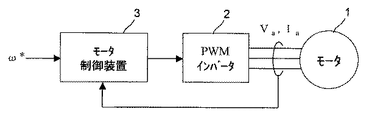•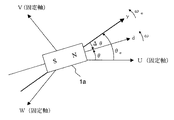••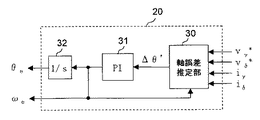•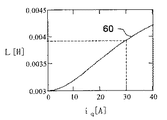•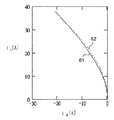•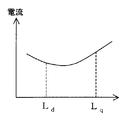•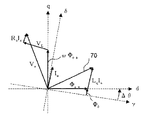•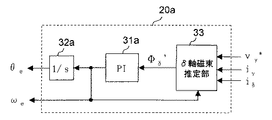•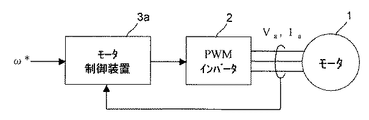•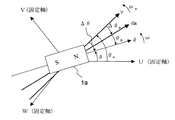•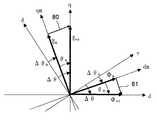•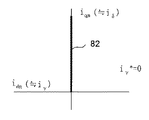•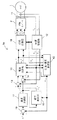•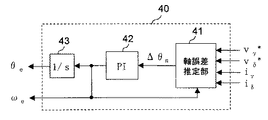•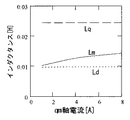•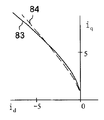•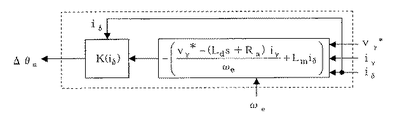•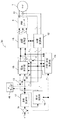•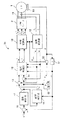•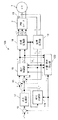•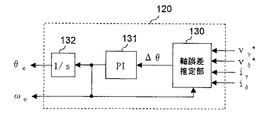•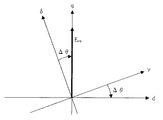•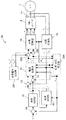•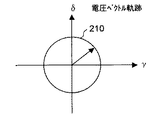•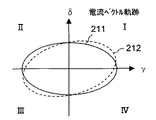••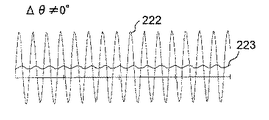•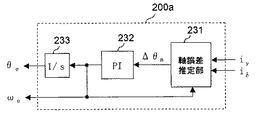•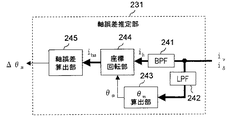••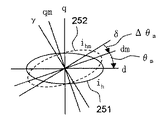•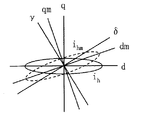•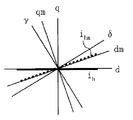•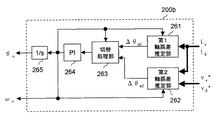•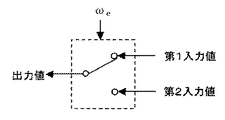••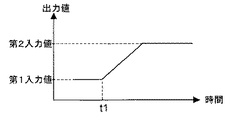•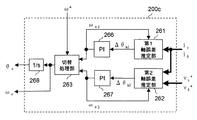•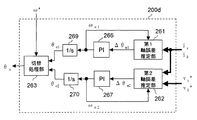## Classifications

• HELECTRICITY
• H02GENERATION; CONVERSION OR DISTRIBUTION OF ELECTRIC POWER
• H02PCONTROL OR REGULATION OF ELECTRIC MOTORS, ELECTRIC GENERATORS OR DYNAMO-ELECTRIC CONVERTERS; CONTROLLING TRANSFORMERS, REACTORS OR CHOKE COILS
• H02P21/00Arrangements or methods for the control of electric machines by vector control, e.g. by control of field orientation
• H02P21/14Estimation or adaptation of machine parameters, e.g. flux, current or voltage
• H02P21/18Estimation of position or speed
• HELECTRICITY
• H02GENERATION; CONVERSION OR DISTRIBUTION OF ELECTRIC POWER
• H02PCONTROL OR REGULATION OF ELECTRIC MOTORS, ELECTRIC GENERATORS OR DYNAMO-ELECTRIC CONVERTERS; CONTROLLING TRANSFORMERS, REACTORS OR CHOKE COILS
• H02P2203/00Indexing scheme relating to controlling arrangements characterised by the means for detecting the position of the rotor
• H02P2203/05Determination of the rotor position by using two different methods and/or motor models
• HELECTRICITY
• H02GENERATION; CONVERSION OR DISTRIBUTION OF ELECTRIC POWER
• H02PCONTROL OR REGULATION OF ELECTRIC MOTORS, ELECTRIC GENERATORS OR DYNAMO-ELECTRIC CONVERTERS; CONTROLLING TRANSFORMERS, REACTORS OR CHOKE COILS
• H02P2203/00Indexing scheme relating to controlling arrangements characterised by the means for detecting the position of the rotor
• H02P2203/09Motor speed determination based on the current and/or voltage without using a tachogenerator or a physical encoder
• HELECTRICITY
• H02GENERATION; CONVERSION OR DISTRIBUTION OF ELECTRIC POWER
• H02PCONTROL OR REGULATION OF ELECTRIC MOTORS, ELECTRIC GENERATORS OR DYNAMO-ELECTRIC CONVERTERS; CONTROLLING TRANSFORMERS, REACTORS OR CHOKE COILS
• H02P2203/00Indexing scheme relating to controlling arrangements characterised by the means for detecting the position of the rotor
• H02P2203/11Determination or estimation of the rotor position or other motor parameters based on the analysis of high frequency signals
• HELECTRICITY
• H02GENERATION; CONVERSION OR DISTRIBUTION OF ELECTRIC POWER
• H02PCONTROL OR REGULATION OF ELECTRIC MOTORS, ELECTRIC GENERATORS OR DYNAMO-ELECTRIC CONVERTERS; CONTROLLING TRANSFORMERS, REACTORS OR CHOKE COILS
• H02P2207/00Indexing scheme relating to controlling arrangements characterised by the type of motor
• H02P2207/05Synchronous machines, e.g. with permanent magnets or DC excitation

## Description

The present invention relates to a motor control device for controlling the operation of a motor. Moreover, it is related with the motor drive system which has this motor control apparatus.

2. Description of the Related Art Conventionally, a motor control device (position sensorless control device) that estimates the rotor position of a motor without using a rotor position sensor and controls the motor based on the estimated rotor position has been developed. FIG. 21 shows an example of a block diagram of this type of motor control device 103. In the configuration shown in FIG. 21, in the motor vector control, the estimated control axis corresponding to the d axis is the γ axis, and the estimated control axis corresponding to the q axis is the δ axis. FIG. 23 shows the relationship among the d-axis, q-axis, γ-axis, and δ-axis. E ex in FIG. 23 is a voltage vector generally called an extended induced voltage.

Current detector 11 detects a motor current supplied from the PWM inverter 2 to the motor 1 of the salient machine U-phase current i u and the V-phase current i v. Coordinate converter 12 converts the U-phase current i u and the V-phase current i v in γ axis current iγ and δ-axis current i?. A position / speed estimator 120 (hereinafter simply referred to as “estimator 120”) estimates and outputs an estimated rotor position θ e and an estimated motor speed ω e .

The subtracter 19 subtracts the estimated motor speed ω e given from the estimator 120 from the motor speed command value ω * and outputs the subtraction result. Based on the subtraction result (ω * −ω e ) of the subtracter 19, the speed control unit 17 creates a δ-axis current command value iδ * that the δ-axis current iδ should follow. The magnetic flux controller 116 outputs a γ-axis current command value iγ * that the γ-axis current iγ should follow based on the δ-axis current command value iδ * and the like. Current controller 15, so that the current error provided via subtracter 13, and 14 (i? * - i?) And current error (i? * - i?) Converge to both zero, the γ-axis voltage value v? * The δ-axis voltage command value vδ * is output.

The coordinate converter 18 performs reverse conversion of the γ-axis voltage command value vγ * and the δ-axis voltage command value vδ * on the basis of the estimated rotor position θ e given from the estimator 120, and the U-phase voltage command value v u. * , Three-phase voltage command values composed of a V-phase voltage command value v v * and a W-phase voltage command value v w * are created and output to the PWM inverter 2. The PWM inverter 2 creates a pulse-width-modulated signal based on the three-phase voltage command values (v u * , v v *, and v w * ), and the motor current corresponding to the three-phase voltage command value. Is supplied to the motor 1 to drive the motor 1.

FIG. 22 shows the internal configuration of the estimator 120. The estimation unit 120 includes an axis error estimation unit 130, a proportional integration calculator 131, and an integrator 132. The axis error estimation unit 130 estimates an axis error Δθ between the d axis and the γ axis. The axis error estimation unit 130 calculates the axis error Δθ using, for example, the following formula (1). Here, L d and L q are a d-axis inductance and a q-axis inductance of the motor 1, respectively, and R a is a motor resistance of the motor 1. S is a Laplace operator. Various methods for estimating the rotor position have been proposed. In the estimation calculation formula, the value of the q-axis inductance of the motor may be used as a calculation parameter as shown in the following formula (1). Many.

The above formula (1) is a formula for calculating the axis error Δθ also shown in Patent Document 1 below. In Patent Document 1 below, the difference between the d axis and the γ axis (dc axis) with respect to the d axis is Δθ, but in this specification, the d axis and the γ axis (dc axis) with the γ axis as a reference. Is treated as Δθ, the sign of the calculation formula of the axis error Δθ and the formula (1) in Patent Document 1 below are reversed. In Expression (1), E ex γ and E ex δ represent the γ-axis component and the δ-axis component of the expansion induced voltage E ex , respectively.

The proportional-plus-integral calculator 131 performs proportional-integral control in cooperation with each part constituting the motor control device 103 to realize a PLL (Phase Locked Loop), and the axis error Δθ calculated by the axis error estimating unit 130. The estimated motor speed ω e is calculated so that the value converges to zero. The integrator 132 calculates the estimated rotor position theta e integrates the estimated motor speed omega e outputted from the proportional-plus-integral calculator 131. The estimated motor speed ω e output from the proportional-plus-integral calculator 131 and the estimated rotor position θ e output from the integrator 132 are both output values of the estimator 120 and each part of the motor control device 103 that requires the values. Given to.

By configuring the motor control device 103 in this way, the axis error Δθ between the d-axis and the γ-axis converges to zero, and stable motor control becomes possible. When the axis error Δθ is maintained at zero, the d-axis current i d follows the γ-axis current command value iγ * , and the q-axis current i q follows the δ-axis current command value iδ *. .

By the way, the calculation formula of the d-axis current i d for performing the maximum torque control using the reluctance torque is widely known. In the motor control device 103 configured as described above, when performing the maximum torque control, the magnetic flux The control unit 116 calculates the γ-axis current command value iγ * based on the following formula (2). Here, Φ a is an armature interlinkage magnetic flux by a permanent magnet.

Patent Document 2 below discloses a position sensorless control method for adjusting the phase of the motor current so that the magnitude of the motor current is minimized.

Non-Patent Document 1 below discloses the relationship between an error in a calculation parameter used for estimating a rotor position and a position estimation error (axis error). Further, motor control techniques using high-frequency voltage or high-frequency current injection are described in Patent Documents 3, 4, 5, and 6 below. Further, a technique related to switching between low-speed sensorless control and high-speed sensorless control is described in Patent Document 7 below.

Japanese Patent No. 3411878 JP 2003-309992 A Shigeo Morimoto and 2 others, "IPMSM Position / Sensorless Control Using Estimated Position Error Information", T.IEE (Electronics D), 2002, Vol. 122, No. 7, p. 722-729 Japanese Patent No. 3312472 JP 2003-219682 A JP 2002-51597 A JP 2003-153582 A Japanese Patent Laid-Open No. 10-94298

In order to realize the maximum torque control using the above equation (2), the axis error Δθ needs to be maintained at zero as a premise. On the other hand, the calculation of the axis error Δθ using the above equation (1) requires the value of the q-axis inductance L q as a calculation parameter (motor parameter). Therefore, conventionally, in order to perform the maximum torque control, the value of the actual q-axis inductance L q of the motor 1 is examined, and the value of the actual q-axis inductance L q is used as it is to determine the axis error Δθ (and thus the estimated rotor position). θ e ) was obtained.

In order to perform high-efficiency operation such as maximum torque control using reluctance torque, it is necessary to flow a d-axis current i d corresponding to the q-axis current i q to the motor, as can be seen from the above equation (2). There is. For this reason, in order to perform such high-efficiency operation, it is necessary to sequentially calculate the γ-axis current command value iγ * .

In addition, the formula for calculating the γ-axis current command value iγ * for performing the maximum torque control has a plurality of motor parameters whose true values are unknown, and these are used for calculating the γ-axis current command value iγ *. If there is an error between the motor parameter (calculation parameter) and the true motor parameter, the desired motor control cannot be performed. For this reason, adjustment for minimizing such an error is essential, but adjustment for a plurality of motor parameters is not easy, and much time is required for the adjustment.

As described above, when performing maximum torque control with a conventional motor control device,
First, it is necessary to adjust parameters (to estimate the rotor position) in order to maintain the axis error Δθ at zero.
Second, it is also necessary to adjust parameters used in the calculation formula (2) for the γ-axis current command value iγ * .
Thirdly, it is necessary to sequentially calculate the γ-axis current command value iγ * requiring complicated calculation.

The parameter adjustment for estimating the rotor position and the parameter adjustment for calculating the γ-axis current command value iγ * are performed separately, and the adjustment time is required accordingly. In addition, the error in the parameter adjustment for estimating the rotor position and the error in the parameter adjustment for calculating the γ-axis current command value iγ * interact with each other, making the adjustment more difficult. In addition, it is difficult to achieve parameter optimization due to the difficulty of adjustment, and as a result, it is difficult to achieve optimal driving of the motor.

Note that the techniques described in Patent Documents 1 and 2 and Non-Patent Document 1 cannot solve the above problems. In Patent Document 2, since an approximation of Δθ≈0 is used, the estimation system decreases as Δθ increases.

Accordingly, an object of the present invention is to provide a motor control device that contributes to facilitating adjustment of calculation parameters for obtaining maximum torque control and / or to reducing the amount of calculation. Moreover, it aims at providing the motor drive system which has such a motor control apparatus.

In order to achieve the above object, a first motor control device according to the present invention is configured such that an axis parallel to a magnetic flux generated by a permanent magnet constituting a rotor is a d axis, and an estimated control axis corresponding to the d axis is a γ axis. An estimator for estimating the rotor position of the motor using a value corresponding to the q-axis inductance of the motor having saliency as a calculation parameter when the axis advanced by 90 degrees in electrical angle from the d-axis is the q-axis And a control unit that controls the motor based on the estimated rotor position, wherein the estimator is between an actual q-axis inductance and an actual d-axis inductance of the motor. Is used as the value of the parameter for calculation, and the rotor position is estimated, thereby causing a deviation between the d-axis and the γ-axis, and the control unit supplies a motor to the motor. Γ-axis of current Min so as to keep a predetermined value of zero or near zero, and controls the motor.

As described above, when the rotor position is estimated, the value between the actual q-axis inductance and the actual d-axis inductance is used as the calculation parameter corresponding to the q-axis inductance without using the actual q-axis inductance. Intentionally, a deviation occurs between the d-axis and the γ-axis. In order to perform maximum torque control or the like, it is necessary to supply a q-axis current corresponding to the d-axis current to the motor. However, since the above-described deviation occurs, the γ-axis component of the motor current is zero or zero. Even if it is kept at a predetermined value in the vicinity, a d-axis current corresponding to the value of the q-axis current actually flows.

That is, with the above configuration, the necessary maximum d-axis current can be obtained only by setting the γ-axis component to a predetermined value near zero or zero without sequentially calculating the value of the γ-axis component of the necessary motor current. Torque control or the like can be realized.

Further, in the conventional example as shown in FIG. 21 and FIG. 22, adjustment of the calculation parameter for rotor position estimation and adjustment of the calculation parameter for maximum torque control are necessary. If configured, the adjustment of the calculation parameters for performing the maximum torque control and the like can be unified with the adjustment of the calculation parameters corresponding to the q-axis inductance. That is, the adjustment of the calculation parameter for obtaining the maximum torque control and the like can be facilitated, and the adjustment time can be reduced. In addition, since it is not necessary to sequentially calculate the value of the γ-axis component of the motor current, the amount of calculation for maximum torque control and the like can be reduced.

Specifically, for example, when an axis advanced 90 degrees in electrical angle from the γ axis is a δ axis, the estimator also estimates the rotation speed of the rotor in response to the estimation of the rotor position, The controller controls the γ-axis current command value and the δ-axis to be followed by the γ-axis component and the δ-axis component of the motor current so that the estimated rotational speed follows the motor speed command value given from the outside. A current command calculation unit for creating a current command value, and the current command calculation unit maintains the γ-axis current command value at the predetermined value regardless of the value of the δ-axis current command value, thereby Regardless of the value of the shaft component, the γ-axis component of the motor current is maintained at the predetermined value.

Further, for example, when the γ-axis component of the motor current is set to the predetermined value and a predetermined load torque is applied to the motor, the calculation parameter is set to a value that gives a minimum value to the magnitude of the motor current. The value of is set.

Thereby, maximum torque control or control approximate to maximum torque control can be obtained.

Further, for example, when the γ-axis component of the motor current is set to the predetermined value and a predetermined load condition is applied to the motor, the calculation parameter is set to a value that gives a minimum value to the loss in the motor. The value is set.

As a result, maximum efficiency control or control approximate to maximum efficiency control can be obtained.

In order to achieve the above object, the second motor control device according to the present invention provides an axis parallel to the magnetic flux generated by the permanent magnet constituting the rotor as the d axis and an estimated axis for control corresponding to the d axis. When an axis advanced 90 degrees in electrical angle from the γ-axis and d-axis is defined as the q-axis, the rotor position of the motor is estimated using a value corresponding to the q-axis inductance of the motor having saliency as a calculation parameter. In the motor control device comprising: an estimator; and a control unit that controls the motor based on the estimated rotor position, the estimator includes an actual q-axis inductance and an actual d-axis inductance of the motor. In this case, the rotor position is estimated after adopting the value between the values as the parameter for calculation, thereby causing a deviation between the d-axis and the γ-axis.

Also by this, realization of the same operation and effect as described above can be expected.

For example, when the actual q-axis inductance and the real d-axis inductance of the motor, respectively and L q and L d, the q-axis inductance as the operation parameter is L, the estimator
L d ≦ L <(L d + L q ) / 2
The rotor position is estimated using L satisfying the above.

Further, for example, the value of the calculation parameter may be a fixed value.

This makes it easier to adjust the operation parameters.

In order to achieve the above object, a third motor control device according to the present invention is a motor control device that controls a motor, and a rotating shaft whose direction coincides with the direction of a current vector when realizing maximum torque control. Alternatively, when the rotation axis whose phase is advanced from the rotation axis is the qm axis and the rotation axis orthogonal to the qm axis is the dm axis, the motor current flowing through the motor is expressed as a qm axis component parallel to the qm axis. The motor is controlled by being decomposed into dm-axis components parallel to the dm-axis.

Even with the configuration described above, it is expected that the calculation parameters can be easily adjusted.

Specifically, for example, in the third motor control device, an estimator that estimates a rotor position of the motor, and a control unit that controls the motor based on the estimated rotor position, When the axis parallel to the magnetic flux generated by the permanent magnet constituting the rotor is the d-axis, the control estimation axis corresponding to the d-axis is the γ-axis, and the axis advanced 90 degrees in electrical angle from the γ-axis is the δ-axis, The control unit controls the motor so that the γ-axis and the δ-axis follow the dm-axis and the qm-axis, respectively.

Further, for example, in the third motor control device, the control unit controls the motor such that the γ-axis component of the motor current is maintained at a predetermined value of zero or near zero.

This eliminates the need for sequential calculation of the value of the γ-axis component of the motor current, thereby reducing the amount of calculation for maximum torque control and the like.

Further, for example, in the third motor control device, the estimator estimates the rotor position using an axial error between the qm axis and the δ axis.

Further, for example, in the third motor control apparatus, when the q-axis is an axis advanced by 90 degrees in electrical angle from the d-axis, the estimator calculates an induced voltage vector on the q-axis generated in the motor. The rotor position is estimated using the induced voltage vector on the qm axis when the induced voltage vector on the qm axis and the induced voltage vector on the dm axis are decomposed.

And for example, in the third motor control device, in the third motor control device, the estimator uses the γ-axis component and the δ-axis component of the induced voltage vector on the qm axis, or the The rotor position is estimated using the γ-axis component of the induced voltage vector on the qm axis.

For example, in the third motor control apparatus, the estimator decomposes the flux linkage vector on the d-axis of the motor into a flux linkage vector on the qm axis and a flux linkage vector on the dm axis. The rotor position is estimated using the flux linkage vector on the dm axis in the case.

For example, in the third motor control device, the estimator uses the γ-axis component and the δ-axis component of the flux linkage vector on the dm axis, or the flux linkage vector on the dm axis. Is used to estimate the rotor position.

Further, for example, in the third motor control device, the control unit converts the predetermined fixed axis component of the motor current into a γ-axis component and a δ-axis component using the rotor position estimated by the estimator. A coordinate converter for converting, and the estimator estimates a qm-axis component and a dm-axis component of the motor current based on a γ-axis component and a δ-axis component of the motor current obtained from the coordinate converter. The rotor position is determined by using an error current between the qm-axis component and dm-axis component of the motor current obtained by estimation and the γ-axis component and δ-axis component of the motor current obtained from the coordinate converter. presume.

Further, for example, in the third motor control device, a superimposition unit that superimposes a superimposed voltage having a frequency different from the driving voltage on a driving voltage for driving the motor is further provided, and the estimator includes the superimposed voltage. The first estimation process for estimating the rotor position is performed based on the superimposed current flowing in the motor in accordance with the superimposition of.

By superimposing a superposition voltage such as a high-frequency rotation voltage and estimating the rotor position based on the superposition current that flows by this superposition, good sensorless control can be realized especially at low speed rotation or rotation stop. It becomes possible.

For example, in the third motor control device, the estimator further includes a second estimation process for estimating the rotor position based on a drive current included in the motor current according to the drive voltage. The estimation process to be actually executed is switched between the first estimation process and the second estimation process in accordance with speed information representing the rotation speed of the rotor.

Thereby, good sensorless control can be realized in a wide speed range.

Specifically, for example, the estimator includes a first candidate axis error calculation unit that calculates an axis error between the qm axis and the δ axis as a first candidate axis error based on the superimposed current; A second candidate axis error calculating unit that calculates an axis error between the qm axis and the δ axis as a second candidate axis error based on the drive current, and estimating the rotor position Is switched between the first candidate axis error and the second candidate axis error according to the speed information, thereby switching between the first estimation process and the second estimation process. Do.

Further, specifically, for example, the estimator is based on a first candidate speed calculation unit that calculates a rotation speed of the rotor as a first candidate speed based on the superimposed current, and on the basis of the drive current. A second candidate speed calculation unit that calculates a rotation speed of the rotor as a second candidate speed, and information used for estimating the rotor position is determined according to the speed information. Switching between the first estimation process and the second estimation process is performed by switching between the candidate speed and the second candidate speed.

Also, specifically, for example, the estimator includes a first candidate position calculation unit that calculates a first candidate position as a candidate for the rotor position to be estimated based on the superimposed current, and the drive A second candidate position calculator that calculates a second candidate position as a candidate for the rotor position to be estimated based on a current, and uses the speed information as information used for estimating the rotor position Accordingly, switching between the first estimation process and the second estimation process is performed by switching between the first candidate position and the second candidate position.

For example, the estimator switches between the first estimation process and the second estimation process that are actually executed according to the speed information or according to the elapsed time from the start of switching. Thus, the estimation process that is actually executed through the estimation process that takes the estimation results of both estimation processes into account is shifted from one estimation process to the other estimation process.

Thereby, smooth switching of the estimation process can be realized.

Further, specifically, for example, the voltage vector locus on the rotation coordinate axis of the superimposed voltage forms a figure having symmetry with the d axis as a reference.

Specifically, for example, when the estimator estimates the rotor position in the first estimation process, the estimator calculates at least one axial component of orthogonal biaxial components that form the vector of the superimposed current. By using it, the rotor position is estimated.

Further, specifically, for example, the estimator includes a coordinate rotation unit that rotates the vector of the superimposed current by a phase difference between the dm axis and the d axis, and the rotation is performed in the first estimation process. When estimating the child position, an axis error between the qm axis and the δ axis is estimated by using at least one of the orthogonal two-axis components forming the current vector obtained by the coordinate rotation, The rotor position is estimated using the axial error.

In order to achieve the above object, a motor drive system according to the present invention includes a motor, an inverter that drives the motor, and the motor control according to any one of the above that controls the motor by controlling the inverter. And a device.

As described above, according to the motor control device and the motor drive system according to the present invention, it is possible to easily adjust the calculation parameters for obtaining the maximum torque control and the like. In addition, the amount of calculation can be reduced.

<< First Embodiment >>
Hereinafter, embodiments of the present invention will be described in detail. First, a first embodiment of the present invention will be described. FIG. 1 is a block diagram of a motor drive system according to the first embodiment. Reference numeral 1 denotes a three-phase permanent magnet synchronous motor 1 (hereinafter simply referred to as “motor 1”) in which a permanent magnet is provided on a rotor (not shown) and an armature winding is provided on a stator (not shown). The motor 1 is a salient pole machine (motor having saliency) typified by an embedded magnet type synchronous motor.

A PWM (Pulse Width Modulation) inverter 2 supplies a three-phase AC voltage composed of a U phase, a V phase, and a W phase to the motor 1 according to the rotor position of the motor 1. The voltage supplied to the motor 1 and the motor voltage (armature voltage) V a, the current supplied from the inverter 2 to the motor 1 the motor current (armature current) and I a.

3 is a motor control device (position-sensorless control device), the motor current I a to estimate the rotor position of the motor 1 by using, PWM inverter a signal for rotating the motor 1 at a desired rotation speed 2 To give. This desired rotation speed is given to the motor control device 3 as a motor speed command value ω * from a CPU (Central Processing Unit) not shown.

FIG. 2 is an analysis model diagram of the motor 1. In the following description, the armature winding refers to that provided in the motor 1. FIG. 2 shows U-phase, V-phase, and W-phase armature winding fixed axes. 1 a is a permanent magnet constituting the rotor of the motor 1. In a rotating coordinate system that rotates at the same speed as the magnetic flux produced by the permanent magnet 1a, the direction of the magnetic flux produced by the permanent magnet 1a is taken as the d-axis, and the estimated control axis corresponding to the d-axis is taken as the γ-axis. Although not shown, the q axis is taken as a phase advanced by 90 degrees in electrical angle from the d axis, and the estimated δ axis is taken as phase advanced by 90 degrees in electrical angle from the γ axis. The rotating coordinate system corresponding to the real axis is a coordinate system in which the d-axis and the q-axis are selected as the coordinate axes, and the coordinate axes are called dq axes. A rotational coordinate system for control (estimated rotational coordinate system) is a coordinate system in which the γ-axis and the δ-axis are selected as coordinate axes, and the coordinate axes are called γ-δ axes.

The dq axes are rotating, and the rotation speed is called the actual motor speed ω. The γ-δ axis is also rotating, and the rotation speed is referred to as an estimated motor speed ω e . In addition, in the dq axes rotating at a certain moment, the phase of the d axis is represented by θ (actual rotor position θ) with reference to the U-phase armature winding fixed axis. Similarly, in the γ-δ axis rotating at a certain moment, the phase of the γ axis is represented by θ e (estimated rotor position θ e ) with respect to the U-phase armature winding fixed axis. Then, (the axis error [Delta] [theta] between the d-q axis and the gamma-[delta] axes) axis error [Delta] [theta] between the d-axis and the gamma-axis is expressed by Δθ = θ-θ e.

In the following description, the γ-axis component, δ-axis component, d-axis component and q-axis component of the motor voltage V a are respectively expressed as γ-axis voltage vγ, δ-axis voltage vδ, d-axis voltage v d and q-axis voltage v q . represents represents γ-axis component of the motor current I a, [delta] -axis component, a d-axis component and q-axis components, respectively γ-axis current i?, [delta] -axis current i?, the d-axis current i d and the q-axis current i q.

In the following description, R a is a motor resistance (resistance value of the armature winding of the motor 1), and L d and L q are d-axis inductances (inductance of the armature winding of the motor 1). d-axis component), q-axis inductance (q-axis component of the inductance of the armature winding of the motor 1), and Φ a is an armature interlinkage magnetic flux by the permanent magnet 1a. Note that L d , L q , R a and Φ a are values determined at the time of manufacturing the motor drive system, and these values are used in the calculation of the motor control device. Moreover, in each formula shown later, s means a Laplace operator.

FIG. 3 is a block diagram showing the configuration of the motor drive system, showing in detail the internal configuration of the motor control device 3 of FIG. The motor control device 3 includes a current detector 11, a coordinate converter 12, a subtractor 13, a subtractor 14, a current control unit 15, a magnetic flux control unit 16, a speed control unit 17, a coordinate converter 18, a subtractor 19, and a position / A speed estimator 20 (hereinafter simply referred to as “estimator 20”) is included. Each part constituting the motor control device 3 can freely use all values generated in the motor control device 3 as necessary.

Current detector 11, for example, a Hall element, detects the U-phase current i u and the V-phase current i v is a fixed axis component of the motor current I a supplied from the PWM inverter 2 to the motor 1. Coordinate converter 12 receives detection results of the U-phase current i u and the V-phase current i v from the current detector 11, with their estimated rotor position theta e fed from the estimator 20, gamma-axis current It converts into iγ and δ-axis current iδ. The following formula (3) is used for this conversion.

The estimator 20 estimates and outputs the estimated rotor position θ e and the estimated motor speed ω e . A method for estimating the estimated rotor position θ e and the estimated motor speed ω e will be described in detail later.

The subtracter 19 subtracts the estimated motor speed ω e given from the estimator 20 from the motor speed command value ω *, and outputs the subtraction result (speed error). The speed control unit 17 creates the δ-axis current command value iδ * based on the subtraction result (ω * −ω e ) of the subtracter 19. The [delta] -axis current value i? * Represents the current value to be followed by the motor current I a [delta] -axis component of a [delta] -axis current i? Is. The magnetic flux controller 16 outputs a γ-axis current command value iγ * . The gamma-axis current value i? * Represents the current value to be followed by the motor current I a gamma-axis component of a gamma-axis current i? Is. As will be described in detail later in relation to the position / speed estimator 20, the γ-axis current command value iγ * is maintained at “zero” in the present embodiment.

The subtracter 13, the γ-axis current value the magnetic flux controller 16 outputs i? *, By subtracting the γ-axis current i? From the coordinate converter 12 outputs, to calculate a current error (i? * - i?). The subtractor 14 subtracts the δ-axis current iδ output from the coordinate converter 12 from the δ-axis current command value iδ * output from the speed control unit 17 to calculate a current error (iδ * −iδ).

The current control unit 15 receives each current error calculated by the subtractors 13 and 14, the γ-axis current iγ and δ-axis current iδ from the coordinate converter 12, and the estimated motor speed ω e from the estimator 20. The γ-axis voltage command value vγ * and the δ-axis voltage command value are set so that the γ-axis current iγ follows the γ-axis current command value iγ * and the δ-axis current iδ follows the δ-axis current command value iδ *.* is output.

The coordinate converter 18 performs inverse conversion of the γ-axis voltage command value vγ * and the δ-axis voltage command value vδ * based on the estimated rotor position θ e given from the estimator 20, and the U phase of the motor voltage V a . Creating a three-phase voltage command value comprising a U-phase voltage command value v u * , a V-phase voltage command value v v * and a W-phase voltage command value v w * representing the component, V-phase component and W-phase component; They are output to the PWM inverter 2. For this inverse transformation, equation (4) consisting of the following two equations is used.

The PWM inverter 2 creates a pulse-width modulated signal based on the three-phase voltage command values (v u * , v v * and v w * ) representing the voltage to be applied to the motor 1, The motor 1 is driven by supplying the motor current I a corresponding to the voltage command value to the motor 1.

FIG. 4 shows an example of the internal configuration of the estimator 20. The estimator 20 of FIG. 4 includes an axis error estimator 30, a proportional-integral calculator 31, and an integrator 32.

The axis error estimation unit 30 calculates an axis error Δθ ′. This axial error Δθ ′ will be apparent from the following description, but is different from the axial error Δθ. 22 calculates the axis error Δθ using the above equation (1), while the axis error estimation unit 30 in FIG. 4 calculates the axis error Δθ ′ using the following equation (5). To do.

Equation (5) is obtained by replacing Δθ and L q in equation (1) with Δθ ′ and L, respectively. For this reason, the axis error estimating unit 30 treats L as a calculation parameter corresponding to the q-axis inductance when estimating the rotor position, and estimates the axis error Δθ ′. The significance of the axis error Δθ ′ in relation to the method for setting the value of the parameter L for calculation and the setting method will be described in detail later.

The proportional-plus-integral calculator 31 performs proportional-integral control in cooperation with each part constituting the motor control device 3 to realize a PLL (Phase Locked Loop), and the axis error Δθ calculated by the axis error estimating unit 30. 'calculates the estimated motor speed omega e to converge to zero. The integrator 32 integrates the estimated motor speed ω e output from the proportional integration calculator 31 to calculate the estimated rotor position θ e . The estimated motor speed ω e output from the proportional integration calculator 31 and the estimated rotor position θ e output from the integrator 32 are both output values of the estimator 20 and each part of the motor control device 3 that requires the values. Given to.

If, in the case of using the true value of the q-axis inductance as L in formula (5) (actual value), i.e., the case of L = L q, [Delta] [theta] '= [Delta] [theta] becomes, PLL control by the proportional-plus-integral calculator 31 etc. As a result, the axis error Δθ ′ (= Δθ) converges to zero (that is, the control is the same as the configuration of FIG. 21). However, as a characteristic point of the present embodiment, the calculation parameter L is set so as to satisfy the following formula (6). That is, after adopting a value between the actual q-axis inductance (that is, L q ) and the actual d-axis inductance (that is, L d ) of the motor 1 as a calculation parameter corresponding to the q-axis inductance, Calculate the error. Of course, L d <L q is satisfied.

Desirably, the calculation parameter L is set so as to satisfy the following expression (7).

The axis error Δθ ′ obtained by adopting L set as described above as a calculation parameter corresponding to the q-axis inductance is naturally different from the axis error Δθ. For this reason, even if the PLL control is performed so that the axis error Δθ ′ converges to zero, a deviation (a non-zero axis error) occurs between the d axis and the γ axis.

In the present embodiment, this deviation is intentionally generated, and the γ-axis current command value iγ * output from the magnetic flux controller 16 is made zero while actively using this deviation, thereby approximating the maximum torque control. Control is performed. This control will be considered below.

First, as disclosed in Non-Patent Document 1, the rotor position estimation (i.e., calculation of the estimated rotor position theta e) of the error and the position estimation error of the calculation parameter used in the (axis error) The relationship is expressed as the following formula (8). Here, R a ′ is the value of the motor resistance as a calculation parameter used in the calculation formula for estimating the rotor position, and (R a −R a ′) is the calculation parameter and the true motor. it represents the error between the resistance R a. L q ′ is a q-axis inductance value as a calculation parameter used in the calculation formula for estimating the rotor position, and (L q −L q ′) is the calculation parameter and the true q-axis inductance. Represents the error.

Now, let L q '= L. That is, assume that an error corresponding to (L q −L) is positively given in estimating the rotor position. Estimating the axis error Δθ ′ using the above equation (5) corresponds to estimating the axis error by positively giving an error corresponding to (L q −L). Further, it is assumed that (R a −R a ′) is zero. Furthermore, as described above, a case is considered where the γ-axis current command value iγ * to be followed by the γ-axis current iγ is zero. That is, in the equation (8), iγ = 0. If it does so, Formula (8) will be transformed like the following formula (9).

Then, substituting equation (10) of the d-axis current i d corresponding to the maximum torque control into equation (9) and solving for L yields the following equation (11). The equation (10) is a generally known equation. If a d-axis current i d satisfying the equation (10) is supplied to the motor 1 according to the q-axis current i q , the maximum torque control is performed. can get.

As is clear from the derivation method of Equation (11), L represented by Equation (11) is an axis for ideally obtaining maximum torque control when the γ-axis current command value iγ * is zero. The value of the q-axis inductance as a calculation parameter to be adopted by the error estimation unit 30 is shown.

L represented by the equation (11) is a function of the q-axis current i q . Hereinafter, for the sake of concrete description, description will be made under numerical examples of Φ a = 0.2411 [Vs / rad], L d = 0.003 [H], L q = 0.008 [H]. . The relationship between i q and L in this case is shown by a curve 60 in FIG. When the γ-axis current command value iγ * is set to zero, the value of L that coincides with the maximum torque control is approximately 0.003 [H] to 0.0042 [A] when 1 [A] ≦ i q ≦ 40 [A]. H]. That is, when the γ-axis electric current command value i? * To zero, the value of L matching the maximum torque control, (in this case, 0.008 [H]) L q quite case of L d (now than, It can be seen that it exists on the side of 0.003 [H]).

Focusing on this, in the present embodiment, a control parameter close to the maximum torque control is adopted by adopting the operation parameter L satisfying the above formula (6) or (7) and setting the γ-axis current command value iγ * to zero. To realize. For example, the above numerical example below, the calculation parameter L regardless i q, L = 0.0039 [H] flowing through the motor 1 when secured to the d-axis current i d and the q-axis current i q The relationship is represented by a broken line 62 in FIG. The solid line 61 is a curve showing the relationship between the d-axis current i d and the q-axis current i q when the maximum torque control is ideally performed. The broken line 62 and the solid line 61 are very similar curves. This can be seen from FIG.

The d-axis current i d corresponding to the q-axis current i q flows even though i γ * = 0, because the calculation satisfies the above formula (6) or (7) as the calculation parameter corresponding to the q-axis inductance. This is because a deviation occurs between the d-axis and the γ-axis due to the use of the parameter L for use. Incidentally, the curve 60 in FIG. 5, when i q = 30 of [A], because that is the L = 0.0039 [H], Naturally is a solid line 61 and broken line 62, i q = 30 [ A].

For the sake of concrete explanation, an example in which the value of the γ-axis current command value iγ * is zero has been described. However, the value of the γ-axis current command value iγ * does not have to be strictly zero, It is only necessary to be a value (i.e., iγ * ≈0). In other words, “zero” in discussing the value of the γ-axis current command value iγ * should be interpreted as “substantially zero” having a certain width. This is because even if iγ * is not strictly zero, control close to maximum torque control can be obtained as long as it can be regarded as substantially zero.

The value of the calculation parameter L is selected from a range that satisfies the above formula (6) or formula (7) in order to realize control similar to the maximum torque control as described above. Specifically, the γ-axis current command value iγ * is set to zero or a predetermined value near zero, thereby setting the γ-axis current iγ to the predetermined value and applying a predetermined load torque to the motor 1. Then, choose in this state, the value of the operation parameter L such as the magnitude of the motor current I a is minimum, from the above equation (6) or within the range satisfying the equation (7). The value of L that gives the minimum value to the magnitude of the motor current I a under i γ * ≈0 exists between L d and L q as shown in FIG. 7, and Φ a , L d , L be employed various values as the value of q, such L satisfies the above expression (7).

When a value of L that gives a minimum value to the magnitude of the motor current Ia is selected under iγ * ≈0, the L is a calculation parameter that ideally realizes maximum torque control at the predetermined load torque. It becomes. The value of the operation parameter L is investigated and set at the design stage.

As described above, by appropriately setting the value of the q-axis inductance as the calculation parameter used for estimating the rotor position, the maximum torque control can be performed only by setting iγ * ≈0 without sequentially calculating iγ *. Control close to can be realized. For this reason, first, an effect of reducing the amount of calculation for maximum torque control is obtained. Further, in the conventional example as shown in FIGS. 21 and 22, adjustment of the calculation parameter for rotor position estimation and adjustment of the calculation parameter for performing the maximum torque control are necessary. The control close to the maximum torque control can be obtained only by adjusting the calculation parameter L for estimating the rotor position. This drastically reduces the time required for adjustment and improves temporal efficiency.

In addition, the example in which the calculation parameter L is a fixed value (L = 0.039 [H] in the above example) regardless of the value of the q-axis current i q has been described above. It may be changed according to the value of the current i q (according to the value of the δ-axis current command value i δ * ). For example, as rests on the curve 60 in FIG. 5, the calculation parameter L (according to the value of δ-axis current value i? *) According to the value of q-axis current i q should be changed, i? * Even when ≈0, ideal maximum torque control can be obtained (in this case, the solid line 61 and the broken line 62 in FIG. 6 completely overlap). Incidentally, depending on the value of q-axis current i q ([delta] in accordance with the axis current value i? * Value) of how to set the operation parameter L, it is sufficient to examine in advance at the design stage.

Further, although the method for obtaining the maximum torque control or the control approximate to the maximum torque control has been described above, other control using the reluctance torque can be obtained depending on the method for setting the calculation parameter L.

For example, the γ-axis current command value iγ * is set to zero or a predetermined value near zero, thereby setting the γ-axis current iγ to the predetermined value and giving a predetermined load condition to the motor 1. In this state, the value of the operation parameter L that minimizes the loss (copper loss and iron loss) in the motor 1 is selected from a range that satisfies the above formula (6) or formula (7). The value of L that gives the minimum value to the loss under iγ * ≈0 exists between L d and L q as in the case of the maximum torque control, and is the value of Φ a , L d , L q Even if various values are adopted, such L satisfies the above formula (7).

When a value of L that minimizes loss under i γ * ≈0 is selected, L becomes a parameter for calculation that realizes maximum efficiency control under the predetermined load condition. The value of the operation parameter L is investigated and set at the design stage. The “predetermined load condition” is, for example, a condition that the motor 1 is rotated at a predetermined rotational speed or a condition that a predetermined load torque is applied to the motor 1.

Further, the current control unit 15 performs necessary calculations using equations (12a) and (12b) including the following two equations. The speed controller 17 and the proportional-plus-integral calculator 31 perform necessary calculations using the following equations (13) and (14), respectively.

Here, K cp , K sp, and K p are proportional coefficients, and K ci , K si, and K i are integral coefficients, which are values set in advance at the time of designing the motor drive system.

The estimation method of the rotor position by the estimator 20 described above is an example, and various estimation methods can be employed. When estimating the rotor position (that is, calculating the estimated rotor position θ e ), any estimation method can be employed as long as the estimation method uses a calculation parameter corresponding to the q-axis inductance of the motor 1. .

For example, the rotor position may be estimated using the method described in Non-Patent Document 1. In the said nonpatent literature 1, axial error (DELTA) (theta) is calculated using following formula (15). When the signs and symbols in the present embodiment are applied, eγ and eδ respectively represent the γ-axis component and δ-axis component of the induced voltage generated by the rotation of the motor 1 and the armature linkage magnetic flux Φ a by the permanent magnet 1a. Represents. Further, s is a Laplace operator, and g is a gain of a disturbance observer.

When the method for estimating the axis error from the induced voltage as shown in the equation (15) is applied to the axis error estimating unit 30 in FIG. 4, the axis error estimating unit 30 uses the following equation (16) to calculate the axis error Δθ. 'Can be calculated. Equation (16) is obtained by replacing Δθ and L q in equation (15) with Δθ ′ and L, respectively. As in the configuration of FIG. 4, the proportional-plus-integral calculator 31 calculates the estimated motor speed ω e and the integrator 32 calculates the estimated rotor position θ e so that the axis error Δθ ′ converges to zero. By doing so, a deviation occurs between the d-axis and the γ-axis.

In addition, the rotor position may be estimated using a method described in Japanese Patent Application Laid-Open No. 2004-96979.

Further, instead of the configuration of FIG. 4, a configuration may be adopted in which the axis error (rotor position) is estimated from the interlinkage magnetic flux that is the source of the induced voltage. A description of this technique will be added. First, the extended induced voltage equation on the real axis is generally expressed as the following equation (17). E ex in the equation (17) is expressed by the equation (18) and is called an extended induced voltage. In the following formula, p is a differential operator.

When the equation (17) on the real axis is coordinate-transformed on the control axis, the equation (19) is obtained.

Further, the magnetic flux when the transient term (second term on the right side) of the equation (18) representing the expansion induced voltage E ex is ignored is defined as the expanded magnetic flux Φ ex as the following equation (20).

By the way, when the motor speed and load are constant, changes in the magnitude and phase of the motor current are very small. Therefore, the second term on the right side of the equation (18), which is the differential term of the q-axis current, is more sufficient than ωΦ ex. Can be regarded as zero. When the motor 1 is driven without step-out, the actual motor speed ω and the estimated motor speed ω e are close to each other, so the third term on the right side of the equation (19) is also sufficiently smaller than ωΦ ex. It can be regarded as zero. Therefore, when ignoring the second term on the right side of the equation (18) and the third term on the right side of the equation (19), the equation (19) becomes the following equation (21).

Here, FIG. 8 shows a vector diagram showing the relationship between the voltages of the respective parts in the motor 1. The motor applied voltage V a is the sum of the expansion induced voltage E ex = ωΦ ex , the voltage drop vector R a · I a at the motor resistance R a , and the voltage drop vector V L at the inductance of the armature winding. expressed. Extended flux [Phi ex is the sum of the magnetic flux (L d -L q) i d to make the magnetic flux [Phi a and d-axis current produced by the permanent magnet, the direction of the vector coincides with the d-axis. A vector represented by L q · I a is a vector of magnetic flux generated by the q-axis inductance and the motor current I a , and reference numeral 70 represents a combined magnetic flux vector of Φ ex and L q · I a .

Further, Faideruta is δ-axis component of the extension magnetic flux [Phi ex. Therefore, Φδ = Φ ex · sin Δθ holds. Further, the following formula (22) is derived by expanding and arranging the first row of the matrix of the formula (21).

Usually, the magnetic flux generated by the permanent magnet is sufficiently larger than the magnetic flux generated by the d-axis current and is Φ a >> (L d −L q ) i d , so Φ ex is constant, that is, Φ ex ≈Φ a Can be considered. If the axial error Δθ is small and can be approximated by sin Δθ≈θ, the following equation (23) is established with reference to the equation (22).

As can be seen from the above equation (23), Φδ is the [delta] -axis component of the armature flux linkage [Phi a (permanent magnet 1a of the motor 1 ([delta] -axis magnetic flux is parallel flux component [delta] axis of FIG. 2)) Approximate to be equal. That is, it is approximated as Φδ≈ (constant value) × Δθ. For this reason, the axial error Δθ converges to zero also by controlling so that Φδ converges to zero. That is, the rotor position and motor speed can be estimated based on Φδ.

Therefore, it is possible to replace the estimator 20 in FIGS. 3 and 4 with an estimator 20a shown in FIG. The estimator 20a includes a δ-axis magnetic flux estimator 33, a proportional-integral calculator 31a, and an integrator 32a. If the axis error Δθ is converged to zero, the δ-axis magnetic flux estimator 33 may estimate the δ-axis magnetic flux Φδ. However, as in the above-described concept, there is an intentional deviation between the d-axis and the γ-axis. In order to generate it, the δ-axis magnetic flux estimation unit 33 estimates the δ-axis magnetic flux Φδ ′ according to the following equation (24). That is, the δ-axis magnetic flux Φδ ′ is calculated using L that satisfies the above formula (6) or formula (7) without using the actual L q as the calculation parameter corresponding to the q-axis inductance.

The proportional-integral calculator 31a is the same as the proportional-integral calculator 31 shown in FIG. 4, and performs proportional-integral control while cooperating with each part constituting the motor control device 3, so that the δ-axis magnetic flux estimating unit 33 The estimated motor speed ω e is calculated so that the calculated δ-axis magnetic flux Φδ ′ converges to zero. The integrator 32a integrates the estimated motor speed ω e output from the proportional integration calculator 31a to calculate the estimated rotor position θ e . The estimated motor speed ω e output from the proportional-plus-integral calculator 31a and the estimated rotor position θ e output from the integrator 32a are both output values of the estimator 20a, and each part of the motor control device 3 that requires these values. Given to.

Incidentally, as can be seen from equation (24), the term including L d since suffering i?, The value of the term is comparatively small. That is, in estimating the rotor position, the influence of the d-axis inductance L d is small (because the value of i γ is much smaller than the value of i δ ). Considering this, L may be used as the value of L d in the equation (24) used for estimation. In this case, the salient pole machine can be operated efficiently with the same control as that used for non-salient pole machines (surface magnet type synchronous motors, etc.) that do not use reluctance torque. There is no need to distinguish and change the control, increasing versatility. Such high versatility can be said also when Expression (5) and Expression (16) are used.

Further, in equation (23), an approximation of Φ ex ≈Φ a is used, but the δ-axis magnetic flux may be estimated without using this approximation. In this case, the δ-axis magnetic flux Φδ ′ may be estimated according to the following formula (25). Also in this case, L that satisfies the above formula (6) or formula (7) is used as the calculation parameter corresponding to the q-axis inductance, without using the actual L q .

<< Second Embodiment >>
Next, a second embodiment of the present invention will be described. In the description of the above-described first embodiment, this embodiment, and other embodiments described later, unless otherwise specified, the same reference numerals denote the same parts and the same symbols (θ, ω, etc.). The ones attached are the same. For this reason, the overlapping description about what attached | subjected the same code | symbol or symbol may be abbreviate | omitted.

FIG. 10 is a block configuration diagram of a motor drive system according to the second embodiment. The motor drive system according to the second embodiment includes a motor 1, an inverter 2, and a motor control device 3a.

The motor control device 3a estimates the rotor position of the motor 1 using the motor current Ia , and gives a signal for rotating the motor 1 at a desired rotational speed to the PWM inverter 2. This desired rotation speed is given as a motor speed command value ω * to the motor control device 3a from a CPU (Central Processing Unit) not shown.

11 and 12 are analysis model diagrams of the motor 1 applied to this embodiment. FIG. 11 shows the U-phase, V-phase, and W-phase armature winding fixed axes. Also in this embodiment, the d-axis, q-axis, γ-axis, and δ-axis, the actual rotor position θ, the estimated rotor position θ e and the axis error Δθ, the actual motor speed ω, and the estimated motor speed ω e are The definition is the same as in the first embodiment (see FIG. 2).

Furthermore, the rotation axis whose direction coincides with the direction of the current vector to be supplied to the motor 1 when realizing the maximum torque control is defined as the qm axis. An axis that is delayed by 90 degrees in electrical angle from the qm axis is defined as the dm axis. A coordinate axis composed of the dm axis and the qm axis is called a dm-qm axis.

As is apparent from the solid line 61 in FIG. 6 representing the current locus when realizing the maximum torque control, the motor current for realizing the maximum torque control has a positive q-axis component and a negative d-axis component. For this reason, the qm axis is an axis whose phase is advanced with respect to the q axis. 11 and 12, the counterclockwise direction is the phase advance direction.

The phase (angle) of the q axis viewed from the qm axis is represented by θ m , and the phase (angle) of the qm axis viewed from the δ axis is represented by Δθ m . In this case, of course, the phase of the d axis seen from the dm axis is also θ m , and the phase of the dm axis seen from the γ axis is also Δθ m . θ m is a lead angle of the qm axis (dm axis) viewed from the q axis (d axis). Δθ m represents an axial error between the qm axis and the δ axis (an axial error between the dm-qm axis and the γ-δ axis). Δθ which is an axial error between the d-axis and the γ-axis is expressed by Δθ = Δθ m + θ m .

As described above, the phase of the dm axis is more advanced than that of the d axis, and θ m takes a negative value at this time. Similarly, when the phase of the γ axis is more advanced than that of the dm axis, Δθ m takes a negative value. The vectors ( Em etc.) shown in FIG. 12 will be described later.

Also, it expressed by the dm-axis and qm-axis components of the motor current I a, respectively, dm-axis current i dm and qm-axis current i qm. The dm-axis and qm-axis components of the motor voltage V a, respectively expressed by the dm-axis voltage v dm and qm-axis voltage v qm.

In the present embodiment, the axis error Δθ m between the qm axis (dm axis) and the δ axis (γ axis) is estimated and the estimated γ axis is converged to the dm axis (that is, the axis error Δθ m is reduced). Converge to zero). Then, the motor 1 is vector-controlled by decomposing the motor current I a into a qm-axis current i qm parallel to the qm-axis and a dm-axis current i dm parallel to the dm-axis.

Again, it is necessary to adjust parameters for estimation (for converging the axis error [Delta] [theta] m to zero) which for estimating the axis error [Delta] [theta] m, for achieving maximum torque control at the same time by making this adjustment The parameter adjustment is completed. That is, since the parameter adjustment for estimating the axis error also serves as the parameter adjustment for realizing the maximum torque control, the adjustment becomes very easy.

As is clear from the definition of the qm-axis current locus of the motor current I a at the time of the maximum torque control is performed, as shown in the solid line 82 in FIG. 13, ride on the qm-axis. Therefore, when performing the maximum torque control, it is not necessary to calculate the complicated γ-axis current command value iγ * as shown in the above equation (2), and the calculation load is reduced. In this case, gamma-axis current value i? * Is set similarly to the first embodiment. That is, for example, the γ-axis current command value iγ * is zero or a predetermined value near zero regardless of the value of iδ.

Next, the significance and specific control method of this embodiment will be described using voltage equations. First, the expansion induced voltage equation on the real axis is expressed by equation (26), and the expansion induced voltage E ex is expressed by equation (27). Equation (26) is the same as equation (17) above, and equation (27) is the same as equation (18) above. In the following formula, p is a differential operator.

When the equation (26) on the real axis is coordinate-transformed on the γ-δ axis that is the estimated axis for control, the equation (28) is obtained, and for the sake of simplicity, the third term on the right side of the equation (28) If ignored, equation (29) is obtained.

Focusing on the dm-qm axis, rewriting equation (29) yields equation (30).

Here, it is defined that Expression (31) is established. Further, considering that i d = i qm · sin θ m , Equation (32) is established.

When Expression (30) is transformed using Expression (32), Expression (33) is obtained. However, Em is represented by Formula (34). L q1 is a virtual inductance that depends on θ m . L q1 is determined for convenience in order to treat E ex · sin θ m existing in the second term on the right side of Equation (30) as a voltage drop due to virtual inductance. L q1 takes a negative value.

Here, it is approximated that the equation: L m = L q + L q1 is established (since θ m depends on i q and i qm , L q1 depends on i q and i qm , and L q also the i q dependence of i q dependent and L q of .L q1 which depends on i q and i qm due to the influence of magnetic saturation, and concentrated in L m, consider the influence of i q and i qm during estimation) . Then, the equation (33) is transformed into the following equation (35). As will be described later, this L m corresponds to the calculation parameter L in the first embodiment.

Further, when the formula (35) is modified, the following formula (36) is obtained. Here, E exm is represented by the following formula (37).

If there is an axis error Δθ m between the γ-δ axis and the dm-qm axis, the equation (36) is transformed as the following equation (38). That is, the equation (38) is obtained by converting the equation (36) on the dm-qm axis to the γ-δ axis in the same manner as the equation (26) is transformed into the equation (28).

Further, when approximated as pΔθ m ≈0, i dm ≈0, and (L d −L q ) (pi q ) ≈0 , E exm represented by Expression (37) is expressed by the following Expression (39). Approximated.

Further, an equation obtained by substituting "L m = L q + L q1" in the above formula (32) is solved for theta m, further, assuming that i? ≒ i qm, the following formula (40) is obtained. As represented by Expression (40), θ m is a function of i δ , so E exm is also a function of i δ .

With reference to FIG. 12, A supplementary description will be given of the relationship between E ex and E m and E exm. Consider E ex , E m and E exm as voltage vectors in the rotating coordinate system. In this case, E ex can be referred to as an extended induced voltage vector. The extended induced voltage vector E ex is an induced voltage vector on the q axis. Consider the extended induced voltage vector E ex into an induced voltage vector on the qm axis and an induced voltage vector on the dm axis. As can be seen from the above equation (34), the induction voltage vector on the qm-axis obtained by this decomposition is E m. Further, the induced voltage vector (E ex · sin θ m ) on the dm-axis, which is obtained by this decomposition and represented by reference numeral 80 in FIG. 12, is a voltage drop vector due to the virtual inductance L q1 .

As can be seen from the comparison between the equations (34) and (37), E exm is obtained by adding ω (L q −L m ) i dm to E m . For this reason, in the rotating coordinate system, E exm is also an induced voltage vector on the qm axis, like E m . When the maximum torque control is performed, since i dm ≈0 as described above, E exm (substantially) matches E m .

Next , the magnetic fluxes corresponding to E ex , E m and E exm will be described with reference to FIG. E ex is an induced voltage generated by Φ ex that is the flux linkage of the motor 1 and the rotation of the motor 1 (see the above formula (20)). Conversely, Φ ex is calculated by dividing E ex by ω (however, the transient term of E ex (the second term on the right side) expressed by equation (27) is ignored).

Given the [Phi ex as a flux linkage vector in a rotating coordinate system, the flux linkage vector [Phi ex is a flux linkage vector on the d-axis. The linkage flux vector Φ ex is considered by being decomposed into an linkage flux vector on the qm axis and an linkage flux vector on the dm axis. If the flux linkage vector on the dm axis obtained by this decomposition is defined as Φ m , Φ m = E m / ω. Further, the flux linkage vector (Φ ex · sin θ m ) on the qm axis, which is obtained by this decomposition and represented by reference numeral 81 in FIG. 12, is a magnetic flux vector due to the virtual inductance L q1 .

If “Φ exm = E exm / ω” is set, Φ exm is obtained by adding (L q −L m ) i dm to Φ m . For this reason, in the rotating coordinate system, Φ exm is also a flux linkage vector on the dm axis, like Φ m . When maximum torque control is performed, i dm ≈0 as described above, and therefore Φ exm (substantially) matches Φ m .

Next, an example of a specific motor drive system using the above equations will be shown. FIG. 14 is a configuration block diagram of the motor drive system showing in detail the internal configuration of the motor control device 3a of FIG. The motor control device 3a includes a current detector 11, a coordinate converter 12, a subtractor 13, a subtractor 14, a current control unit 15, a magnetic flux control unit 16, a speed control unit 17, a coordinate converter 18, a subtractor 19, and a position / A speed estimator 40 (hereinafter simply referred to as “estimator 40”) is included. That is, the motor control device 3a in FIG. 14 has a configuration in which the estimator 20 in the motor control device 3 in FIG. Each part constituting the motor control device 3a can freely use all the values generated in the motor control device 3a as necessary.

Current detector 11 detects the U-phase current i u and the V-phase current i v is a fixed axis component of the motor current I a. Coordinate converter 12 receives detection results of the U-phase current i u and the V-phase current i v from the current detector 11, with their estimated rotor position theta e fed from the estimator 40, gamma-axis current It converts into iγ and δ-axis current iδ. For this conversion, the above equation (3) is used as in the first embodiment.

The estimator 40 estimates and outputs the estimated rotor position θ e and the estimated motor speed ω e . A specific estimation method by the estimator 40 will be described later.

The subtracter 19 subtracts the estimated motor speed ω e given from the estimator 40 from the motor speed command value ω * and outputs the subtraction result (speed error). The speed control unit 17 creates the δ-axis current command value iδ * based on the subtraction result (ω * −ω e ) of the subtracter 19. The magnetic flux controller 16 outputs a γ-axis current command value iγ * . The γ-axis current command value iγ * is set in the same manner as in the first embodiment as described above. For example, iγ * is zero or a predetermined value near zero.

The subtracter 13, the γ-axis current value the magnetic flux controller 16 outputs i? *, By subtracting the γ-axis current i? From the coordinate converter 12 outputs, to calculate a current error (i? * - i?). The subtractor 14 subtracts the δ-axis current iδ output from the coordinate converter 12 from the δ-axis current command value iδ * output from the speed control unit 17 to calculate a current error (iδ * −iδ).

The current control unit 15 receives each current error calculated by the subtractors 13 and 14, the γ-axis current iγ and δ-axis current iδ from the coordinate converter 12, and the estimated motor speed ω e from the estimator 40. The γ-axis voltage command value vγ * and the δ-axis voltage command value are set so that the γ-axis current iγ follows the γ-axis current command value iγ * and the δ-axis current iδ follows the δ-axis current command value iδ *.* is output.

Coordinate converter 18, based on the estimated rotor position theta e fed from the estimator 40 performs inverse transformation of the γ-axis voltage value v? * And the δ-axis voltage command value vδ *, v u *, v v * And v w * are generated, and these are output to the PWM inverter 2. For this inverse transformation, the above equation (4) is used as in the first embodiment. The PWM inverter 2 drives the motor 1 by supplying a motor current I a corresponding to the three-phase voltage command value to the motor 1.

FIG. 15 shows an example of the internal configuration of the estimator 40. The estimator 40 in FIG. 15 includes an axis error estimator 41, a proportional-plus-integral calculator 42, and an integrator 43. The proportional-integral calculator 42 and the integrator 43 are the same as the proportional-integral calculator 31 and the integrator 32 in FIG. 4, respectively.

Axis error estimator 41, vγ *,*, calculates the axis error [Delta] [theta] m by using all or part of the value of iγ and i?. The proportional-plus-integral computing unit 42 performs proportional-integral control in cooperation with each part constituting the motor control device 3a to realize a PLL (Phase Locked Loop), and the axis error Δθ calculated by the axis error estimating unit 41. The estimated motor speed ω e is calculated so that m converges to zero. The integrator 43 integrates the estimated motor speed ω e output from the proportional integration calculator 42 to calculate the estimated rotor position θ e . The estimated motor speed ω e output from the proportional-integral calculator 42 and the estimated rotor position θ e output from the integrator 43 are both output values of the estimator 40, and each part of the motor control device 3a that requires the value. Given to.

As method for calculating the axis error [Delta] [theta] m by the axial error estimator 41, it can be applied a variety of calculation methods. Hereinafter, as a calculation method of the axis error Δθ m by the axis error estimation unit 41 (in other words, as a calculation method of θ e by the estimator 40), the first, second, third, fourth, and fifth calculation methods are described. Is illustrated.

The axis error estimation unit 41 uses the values of vγ * , vδ *, and ω e as the values of vγ, vδ, and ω in the respective equations when using the equations described in this specification. . The contents described in each calculation method (such as a method for determining the value of L m ) can be applied to other calculation methods and all other embodiments described later.

[First calculation method]
First, a first calculation method of the axis error Δθ m will be described. In the first calculation method, the induced voltage E ex generated in the motor 1 is considered by being decomposed into an induced voltage vector on the qm axis and an induced voltage vector on the dm axis. Then, an axis error Δθ m is calculated using an induced voltage vector E exm ( ≈E m ; see FIG. 12) that is an induced voltage vector on the qm axis, and thereby the phase of the γ axis that is an estimated axis for control. (Θ e ) is calculated (ie, the rotor position is estimated).

Assuming that E exm γ and E exm δ are the γ-axis component and δ-axis component of the induced voltage vector E exm , respectively, Δθ m = tan −1 (−E exm γ / E exm δ ) Holds. Then, using the result of transforming the first and second rows of the determinant (38), Δθ m is expressed as the following equation (41) (however, the right side of the determinant (38)) Ignore the third term). In the equation (41), assuming that Δθ m is finally small, an approximation of tan −1 (−E exm γ / E exm δ) ≈ (−E exm γ / E exm δ) is used. .

The axis error estimator 41 can ignore the differential terms pL d iγ and pL d iδ when calculating Δθ m using Equation (41). Further, the following formula (42) is used to calculate the value of L m necessary for calculating Δθ m . The equation obtained by substituting “i dm = 0 and the following equations (43) and (44)” into the equation (32) is solved for L q1 , and the result is used to obtain the equation (42). be able to.

Furthermore, the above equation (45) using the equation (45) of the d-axis current i d corresponding to the maximum torque control and the equation (43) which is a relational equation (approximate equation) of i d , i q, and i qm ( 42), L m becomes a function of i qm (that is, the terms of i d and i q disappear from the calculation formula of L m ). Therefore, the axis error estimation unit 41 can calculate the value of L m represented by a function of i qm based on i δ by assuming that i δ ≈ i qm . Then, the axis error Δθ m is calculated from the equation (41) using the calculated value of L m .

Table Incidentally, assuming that i? ≒ i qm, the L m to may be to obtain the values of L m by using an approximate expression expressed as a function of i?, The value of L m corresponding to i? Beforehand A value of L m may be obtained by preparing as data and referring to the table data.

FIG. 16 shows a graph under a certain numerical example showing the dependence of L d , L q, and L m on i qm (assuming i γ * ≈0). As shown in FIG. 16, the value of L m is dependent on i qm, increases as i qm increases. L m which defines in this embodiment is equivalent to the calculation parameter L in the first embodiment, the value of L m matching the maximum torque control, L similar, L q much L d than (See also FIGS. 5 and 7).

As a result, the value of L m is determined so as to satisfy the following formula (46) or formula (47), as in the first embodiment. As a result, the motor control device 3a according to the present embodiment intentionally causes a deviation between the d-axis and the γ-axis and sets iγ * ≈0, as in the first embodiment, so that the maximum torque control is performed. Control close to is realized.

L m may be a fixed value. That is, a fixed value regardless of the value of iδ may be adopted as the value of L m . The relationship between the d-axis current i d and the q-axis current i q when L m is a predetermined fixed value is represented by a solid line 83 in FIG. A broken line 84 is a curve showing a relationship between the d-axis current i d and the q-axis current i q when the maximum torque control is ideally performed, but the solid line 83 and the broken line 84 are very similar curves. This can be seen from FIG.

[Second calculation method]
Next, a second calculation method of the axis error Δθ m will be described. Also in the second calculation method, the axis error Δθ m is calculated using the induced voltage vector E exm in the same manner as in the first calculation method described above, and thereby the phase (θ e ) of the γ axis, which is an estimation axis for control. Is calculated (ie, the rotor position is estimated). However, in the second calculation method, the δ-axis component E exm δ of the induced voltage vector E exm is not used. Specifically, the axis error Δθ m is calculated using the following equation (48). In equation (48), an approximation of sin −1 (−E exm γ / E exm ) ≈ (−E exm γ / E exm ) is used assuming that Δθ m is finally small.

The axis error estimator 41 can ignore the differential term pL d iγ when calculating Δθ m using Equation (48). Further, the value of L m is determined by a method similar to the method in the first calculation method.

For calculating E exm in the equation (48), the above equation (39) is used. As an approximate expression for calculating E exm , for example, the following expression (49), (50), or (51) can be used. Expression (49) is an approximation expression of Expression (37) using an approximation of “pΔθ m ≈0, i dm ≈0, (L d −L q ) (pi q ) ≈0”, and Expression (50) is Furthermore, it is an approximate expression of the expression (49) using the approximation of “cos θ m ≈1”, and the expression (51) is an expression using an approximation of “(L d −L q ) iδsin θ m << Φ a ” ( 50). Note that ω e is used as the value of ω when using the formula (49), (50), or (51).

In order to calculate θ m included in the equation (49) and the like, the above equation (40) is used. As can be seen from equation (40), θ m is a function of i δ , so E exm is also a function of i δ . Since the calculation of E exm is complicated, it is desirable to use an appropriate approximate expression for the calculation. It is also possible to prepare the value of E exm corresponding to as table data in advance and obtain the value of E exm by referring to the table data.

[Third calculation method]
Next, a third calculation method for the axial error Δθ m will be described. In the third calculation method, the linkage flux Φ ex that links the armature windings of the motor 1 is considered to be broken down into a linkage flux vector on the qm axis and a linkage flux vector on the dm axis. Then, an axis error Δθ m is calculated using an interlinkage magnetic flux vector Φ exm ( ≈Φ m ; see FIG. 12) which is an interlinkage magnetic flux vector on the dm axis. The axis phase (θ e ) is calculated (ie, the rotor position is estimated).

The gamma-axis component and [delta] -axis component of the flux linkage vector [Phi exm, respectively, when [Phi exm gamma and [Phi exm [delta], as is clear from FIG. 12, Δθ m = tan -1 ( -Φ exm δ / Φ exm γ) holds. Since Φ exm is obtained by dividing E exm by ω, Δθ m is expressed by the following equation (52). In equation (52), assuming that Δθ m is finally small, an approximation of tan −1 (−Φ exm δ / Φ exm γ) ≈ (−Φ exm δ / Φ exm γ) is used. .

The axis error estimator 41 can ignore the differential terms pL d iγ and pL d iδ when calculating Δθ m using Equation (52). Further, the value of L m is determined by a method similar to the method in the first calculation method.

[Fourth calculation method]
Next, a fourth calculation method for the axial error Δθ m will be described. In the fourth calculation method, as in the third calculation method described above, the axis error Δθ m is calculated using the flux linkage vector Φ exm , and thereby the phase of the γ axis (θ e), which is an estimated axis for control, is calculated. ) Is calculated (ie, the rotor position is estimated). However, in the fourth calculation method does not utilize gamma-axis component of the flux linkage vector Φ exm Φ exm γ. Specifically, the axis error Δθ m is calculated using the following equation (53). In equation (53), assuming that Δθ m is finally small, an approximation of sin −1 (−Φ exm δ / Φ exm ) ≈ (−Φ exm δ / Φ exm ) is used.

The axis error estimator 41 can ignore the differential term pL d iγ when calculating Δθ m using Equation (53). Further, the value of L m is determined by a method similar to the method in the first calculation method.

For calculating Φ exm in the equation (53), an equation obtained by dividing both sides of the equation (39) by ω is used. As an approximate expression for calculating Φ exm , for example, the following expression (54), (55), or (56) can be used. The following formulas (54), (55), and (56) are formulas obtained by dividing both sides of the formulas (49), (50), and (51) by ω, respectively. Note that ω e is used as the value of ω when using the formula (54), (55), or (56).

To calculate the theta m included in formula (54) or the like, the formula (40) is utilized. As can be seen from the equation (40), θ m is a function of i δ , so that Φ exm is also a function of i δ . Since calculation of Φ exm is complicated, it is desirable to use an appropriate approximate expression for the calculation. It is also possible to prepare the value of Φ exm corresponding to as table data in advance and obtain the value of Φ exm by referring to the table data.

If K ( ) = 1 / Φ exm and K ( ) is regarded as a correction coefficient, the internal configuration of the axis error estimation unit 41 in the fourth calculation method is as shown in FIG. Further, instead of using the correction coefficient K (iδ), the gain (proportional coefficient or integral coefficient) used in the proportional integration calculator 42 may be changed according to the value of iδ.

[Fifth calculation method]
Next, a fifth calculation method for the axis error Δθ m will be described. In the fifth calculation method, the axis error Δθ m is calculated by using the error current between the current on the dm-qm axis (current of the motor model) and the current on the γ-δ axis, and thereby estimation for control is performed. axis in a γ-axis phase (theta e) is calculated (i.e., the rotor position is estimated).

This technique will be described using mathematical expressions. First, if the third term on the right side of the above equation (38) is ignored, the following equation (57) is obtained.

When discretized with the sampling period T s , Expression (57) can be written as Expression (58) below.

On the other hand, the estimated currents i M γ and i M δ obtained by the calculation of the axis error estimator 41 use estimated induced voltages E Mexm γ and E Mexm δ obtained by modeling E exm γ and E exm δ as models, It is represented by the following formula (59).

Axis error estimator 41 as an estimate of E exm gamma and E exm [delta], to calculate the estimated induced voltage E Mexm γ and E Mexm δ respectively. Since the estimated currents i M γ and i M δ are calculated using L m instead of L q , the estimated currents i M γ and i M δ are the dm-axis component and qm of the motor current I a , respectively. It can be referred to as a current estimated axis component.

The difference between the current iγ and iδ based on fixed-axis components of the motor current I a detected by the current detector 11 (i u and i v), and the estimated current i M gamma and i M [delta] was obtained by calculation, Certain error currents Δiγ and Δiδ are expressed by the following equation (60) from equations (58) and (59).

Here, Delta] E exm gamma, is the error between the estimated induced voltage E Mexm gamma is an estimated value of the induced voltage E exm gamma and the induced voltage E exm γ, ΔE exm δ is induced voltage E exm [delta] and the induced voltage E This is an error from the estimated induced voltage E Mexm δ which is an estimated value of exm δ.

As apparent from the equation (60), the error (ΔE exm γ, etc.) of the estimated value of the induced voltage and the error current (Δiγ, etc.) are in a proportional relationship. For this reason, the error of the estimated value of the induced voltage can be converged using the error current. That is, the estimated induced voltages E Mexm γ and E Mexm δ can be used as correctly estimated induced voltages E exm γ and E exm δ (the induced voltage can be correctly estimated).

Specifically, the current estimated induced voltage is calculated using the previous estimated induced voltage and the previous estimated error. More specifically, the estimated induced voltages E Mexm γ and E Mexm δ are sequentially calculated by the following equation (61). Here, g is a field back gain for converging the error of the estimated value of the induced voltage.

Then, as in the first or second calculation method described above, the axis error estimation unit 41 calculates the axis error Δθ m using the following formula (62) or (63).

In the equations (58) to (63), the symbol (n or n−1) written in parentheses “()” represents the sampling timing when discretized with the sampling period T s . n is a natural number, and n represents a sampling timing that comes next to (n-1). Each portion constituting the motor control device 3a, for each sampling period T s, successive, calculates and outputs the values. Specifically, for example, iγ (n) and iδ (n) are iγ and iδ at the nth sampling timing, and iγ (n−1) and iδ (n−1) are (n−1). I γ and i δ at the first sampling timing. The same applies to other than iγ and iδ.

As described above, in this embodiment, the axis error Δθ m is converged to zero, and the γ axis is made to follow the dm axis. As a result, i γ and i δ follow i dm and i qm , respectively. That is, it can be said that the motor control device 3a performs drive control of the motor 1 by decomposing the current flowing through the motor 1 into a qm-axis component and a dm-axis component. The effects obtained by this decomposition are as described above.

<< Third Embodiment >>
Further, the configuration of the motor control device 3a shown in FIG. 14 may be modified as in the motor control device 3b of FIG. The modified embodiment is a third embodiment of the present invention. The motor control device 3b has a configuration in which the estimator 40 of the motor control device 3a in FIG. 14 is replaced with a position / speed estimator 45 (hereinafter abbreviated as an estimator 45), a θ m calculation unit 46, and a calculator 47. It has become. Except for the replacement, the motor control device 3a and the motor drive system in FIG. 14 are the same as the motor control device 3b and the motor drive system in FIG. A description of the configuration and operation of similar parts is omitted.

The estimator 45 uses iγ, iδ, vγ *, and vδ * to estimate the d-axis phase viewed from the U phase, and outputs the estimated value as θ dqe . The estimator 45 also calculates the estimated motor speed ω e as with the estimator 40 in the second embodiment. When the estimated motor speed ω e output from the estimator 45 is obtained by differentiating θ dqe , the obtained estimated motor speed ω e should be accurately called an estimated value of the d-axis rotational speed. However, in the steady state, the estimated value and ω e that is the rotational speed of the γ-axis can be regarded as the same.

theta m calculator 46, while using the i? * from the speed controller 17 as i? in the formula (40), calculates the theta m by using equation (40). At this time, a value of θ m corresponding to i δ * may be prepared in advance as table data, and the value of θ m may be obtained by referring to the table data.

Calculator 47 calculates the theta e using theta m outputted from the estimator 45 and theta DQE output from theta m calculator 46, giving a calculated theta e in the coordinate converter 12 and 18.

As described above, in the third embodiment, the part constituted by the estimator 45, the θ m calculation unit 46, and the calculator 47 calculates the phase (θ e ) of the γ axis that is the control estimation axis. Become. Even if it comprises like 3rd Embodiment, the effect | action and effect similar to 2nd Embodiment can be acquired.

<< Fourth Embodiment >>
Moreover, although the 1st-3rd embodiment employ | adopts the system which provides an estimator and estimates a rotor position, you may make it detect an actual rotor position. That is, instead of the motor control device shown in FIG. 3, FIG. 14 or FIG. 19, the motor control device 3c of FIG. 20 may be used.

A motor drive system including the motor control device 3c shown in FIG. 20 will be described as a fourth embodiment of the present invention. FIG. 20 is a configuration block diagram of a motor drive system according to the fourth embodiment. The motor drive system includes a motor 1, an inverter 2, and a motor control device 3c.

The motor control device 3c includes a current detector 11, a coordinate converter 12, a subtractor 13, a subtractor 14, a current control unit 15, a magnetic flux control unit 16, a speed control unit 17, a coordinate converter 18, a subtractor 19, and a position detection. A calculator 50, a differentiator 51, a θ m calculator 52, and a calculator 53. That is, the motor control device 3c has a configuration in which the estimator 40 in FIG. 14 is replaced with “a position detector 50, a differentiator 51, a θ m calculation unit 52, and a calculator 53”. Except for the replacement, the motor control device 3a and the motor drive system in FIG. 14 are the same as the motor control device 3c and the motor drive system in FIG. Each part constituting the motor control device 3c can freely use all the values generated in the motor control device 3c as necessary.

Since each part in the motor control device 3c operates based on the detected actual rotor position instead of the estimated rotor position, in this embodiment, “γ and δ” in the second embodiment is “dm and qm”. "

The position detector 50 is composed of a rotary encoder or the like, detects the actual rotor position θ of the motor 1, and gives the value to the differentiator 51 and the calculator 53. The differentiator 51 differentiates the actual rotor position θ to calculate the actual motor speed ω, and gives the value to the subtractor 19, the magnetic flux controller 16 and the current controller 15.

In the steady state, the actual motor speed ω and the rotational speed of the dm-qm axis can be regarded as the same. For this reason, the input value of the differentiator 51 is θ, but the input value of the differentiator 51 may be the output value θ dm of the calculator 53 instead of θ.

The speed control unit 17 creates a qm-axis current command value i qm * that the qm-axis current i qm should follow based on the subtraction result (ω * −ω) of the subtracter 19. The magnetic flux controller 16 outputs a dm-axis current command value i dm * that the dm-axis current i dm should follow. This dm-axis current command value i dm * is set in the same manner as in the second embodiment. That is, for example, i dm * is set to zero or a predetermined value near zero.

The subtractor 13 calculates a current error (i dm * −i dm ) by subtracting i dm output from the coordinate converter 12 from i dm * output from the magnetic flux controller 16. Subtractor 14, from i qm * outputted by the speed controller 17 subtracts the i qm from the coordinate converter 12 outputs, to calculate a current error (i qm * -i qm).

The current control unit 15 receives each current error calculated by the subtracters 13 and 14, i dm and i qm from the coordinate converter 12, and the actual motor speed ω from the differentiator 51, and i dm becomes i dm * a so as to follow, and so i qm follows the i qm *, the v dm dm-axis voltage command value should follow v dm * and v qm to be followed qm-axis voltage command value v qm * Output.

theta m calculator 52 while using the i qm * from the speed controller 17 as iδ in the above formula (40), calculates the theta m by using equation (40). In this case, it may be to obtain the value of theta m by referring to the i qm * (iδ *) the table data is prepared as table data the value of theta m in advance in accordance with the.

Calculator 53, by using the theta m calculated by theta and theta m calculator 52 detected by the position detector 50 calculates the phase theta dm of dm-axis viewed from the U-phase, calculated theta dm Is provided to coordinate converters 12 and 18.

The coordinate converter 18 converts v dm * and v qm * into a three-phase voltage command value composed of v u * , v v * and v w * based on a given θ dm and is obtained by conversion. The obtained value is output to the PWM inverter 2. The PWM inverter 2 drives the motor 1 by supplying a motor current I a corresponding to the three-phase voltage command value to the motor 1.

Even if it constitutes like a 4th embodiment, the same operation and effect as a 2nd embodiment can be acquired.

<< Fifth Embodiment >>
Incidentally, the sensorless control described in the second and third embodiments (FIGS. 14 and 19) is a control based on the generated induced voltage and the like, and is particularly useful when the motor 1 rotates at high speed. However, the accuracy of estimation is not always sufficient when rotating at low speed, and it cannot be applied when rotation is stopped. In the fifth embodiment, sensorless control based on the dm-qm axis that functions particularly effectively during low-speed rotation or rotation stop will be described.

FIG. 24 is a block diagram of a motor control device 3d according to the fifth embodiment. The motor control device 3d includes a current detector 11, a coordinate converter 12, a subtractor 13, a subtractor 14, a current control unit 15, a magnetic flux control unit 16, a speed control unit 17, a coordinate converter 18, a subtractor 19, and a position / The apparatus includes a speed estimator 200 (hereinafter simply referred to as “estimator 200”), a superimposed voltage generation unit 201, an adder 202, and an adder 203. Each part constituting the motor control device 3d can freely use all the values generated in the motor control device 3d as necessary.

In the motor control device 3d, a superimposed voltage generation unit 201 and adders 202 and 203 are newly added, and the estimator 40 in the motor control device 3a in FIG. 14 is different from the motor control device 3a of FIG. 14, and the motor control devices 3d and 3a are the same in other points. Note that the matters described in the second embodiment are applied to the present embodiment as long as there is no contradiction.

The estimator 200 estimates and outputs the estimated rotor position θ e and the estimated motor speed ω e . A specific estimation method by the estimator 200 will be described later. Coordinate converter 12, the detected U-phase current i u and the V-phase current i v by the current detector 11, by using the estimated rotor position theta e fed from the estimator 200, gamma-axis current iγ and δ Convert to shaft current iδ.

The subtracter 19 subtracts the estimated motor speed ω e given from the estimator 200 from the motor speed command value ω *, and outputs the subtraction result (speed error). The speed control unit 17 creates the δ-axis current command value iδ * based on the subtraction result (ω * −ω e ) of the subtracter 19. The magnetic flux controller 16 outputs a γ-axis current command value iγ * . This γ-axis current command value iγ * is set in the same manner as in the first embodiment. For example, iγ * is zero or a predetermined value near zero.

The subtracter 13, the γ-axis current value the magnetic flux controller 16 outputs i? *, By subtracting the γ-axis current i? From the coordinate converter 12 outputs, to calculate a current error (i? * - i?). The subtractor 14 subtracts the δ-axis current iδ output from the coordinate converter 12 from the δ-axis current command value iδ * output from the speed control unit 17 to calculate a current error (iδ * −iδ).

The current control unit 15 receives each current error calculated by the subtracters 13 and 14, the γ-axis current iγ and δ-axis current iδ from the coordinate converter 12, and the estimated motor speed ω e from the estimator 200. The γ-axis voltage command value vγ * and the δ-axis voltage command value are set so that the γ-axis current iγ follows the γ-axis current command value iγ * and the δ-axis current iδ follows the δ-axis current command value iδ *.* is output.

The superimposed voltage generation unit 201 generates and outputs a superimposed voltage to be superimposed on the γ-axis voltage command value vγ * and the δ-axis voltage command value vδ * . The superposed voltage is, v? * With respect to gamma-axis superposed voltage v h gamma * and (gamma-axis component of the superposed voltages), v? * With respect to [delta] axis superposed voltage v h [delta] * and ([delta] -axis component of the superposed voltage) it consists. Hereinafter, the γ-axis superimposed voltage v h γ * and the δ-axis superimposed voltage v h δ * may be collectively referred to as the superimposed voltage v h γ * and v h δ * .

The adder 202 adds the γ-axis superimposed voltage v h γ * to the γ-axis voltage command value vγ * output from the current control unit 15, and sends the addition result (vγ * + v h γ * ) to the coordinate converter 18. Output. The adder 203 adds the δ-axis superimposed voltage v h δ * to the δ-axis voltage command value vδ * output from the current control unit 15, and the addition result (vδ * + v h δ * ) is sent to the coordinate converter 18. Output.

The coordinate converter 18 is based on the estimated rotor position θ e given from the estimator 200, and a γ-axis voltage command value on which v h γ * is superimposed (that is, (vγ * + v h γ * )) and v h. Performs inverse transformation of the δ-axis voltage command value (ie, (vδ * + v h δ * )) on which δ * is superimposed to create three-phase voltage command values (v u * , v v *, and v w * ) Then, they are output to the PWM inverter 2. For this inverse transformation, equations are used in which vγ * and vδ * in equation (4) are replaced with (vγ * + v h γ * ) and (vδ * + v h δ * ), respectively. The PWM inverter 2 drives the motor 1 by supplying a motor current I a corresponding to the three-phase voltage command value to the motor 1.

Thus, the superimposed voltage is superimposed on the drive voltage for driving the motor 1 represented by vγ * and vδ * . By superimposing the superimposed voltage, the superimposed current corresponding to the superimposed voltage is superimposed on the drive current for driving the motor 1 represented by the γ-axis current command value iγ * and the δ-axis current command value iδ * . Will be.

The superimposed voltage generated by the superimposed voltage generation unit 201 is, for example, a high-frequency rotation voltage. Here, “high frequency” means that the frequency of the superimposed voltage is sufficiently larger than the frequency of the drive voltage. Therefore, the frequency of the superimposed current superimposed according to the superimposed voltage is sufficiently larger than the frequency of the drive current. “Rotational voltage” means a voltage such that the voltage vector locus of the superimposed voltage forms a circle on the fixed coordinate axis.

Even when considered on a rotational coordinate axis such as the dq axis or the γ-δ axis, the voltage vector locus of the superimposed voltage generated by the superimposed voltage generation unit 201 is, for example, a circle like the voltage vector locus 210 in FIG. Make it. When the superposed voltage is a three-phase balanced voltage, the voltage vector locus forms a perfect circle centered on the origin on the rotation coordinate axis as the voltage vector locus 210. Since this rotation voltage (superimposed voltage) is a high-frequency voltage that is not synchronized with the motor 1, the application of this rotation voltage does not cause the motor 1 to rotate.

In addition, when the motor 1 is an embedded magnet type synchronous motor or the like and L d <L q is satisfied, the current vector locus of the superimposed current flowing in the motor 1 by the superimposed voltage forming the voltage vector locus 210 is the current in FIG. As indicated by a vector locus 211, an ellipse is formed on the γ-δ axis with the origin at the center, the γ-axis direction as the major axis direction, and the δ-axis direction as the minor axis direction. However, the current vector locus 211 is a current vector locus when the axis error Δθ between the d-axis and the γ-axis is zero. When the axial error Δθ is not zero, the current vector locus of the superimposed current is an ellipse represented by the current vector locus 212, and the major axis direction does not coincide with the γ-axis direction. That is, when the axis error Δθ is not zero, the current vector locus 211 is tilted about the origin on the γ-δ axis, and the current vector locus 212 is drawn.

Assuming that the γ-axis component and δ-axis component of the superimposed current are the γ-axis superimposed current i h γ and the δ-axis superimposed current i h δ, respectively, the product (i h γ × i h δ) has a current vector locus 212. There is a DC component that depends on the slope of the ellipse represented by. When the product (i h γ × i h δ) takes a positive value in the first and third quadrants of the current vector locus and takes a negative value in the second and fourth quadrants, the ellipse is not inclined. Does not include a DC component (in the case of the current vector locus 211), but includes an DC component when the ellipse is tilted (in the case of the current vector locus 212). In FIG. 26, I, II, III, and IV represent the first, second, third, and fourth quadrants on the γ-δ axis.

In FIG. 27, time is plotted on the horizontal axis, and the product (i h γ × i h δ) and the DC component of the product when the axis error Δθ is zero are represented by curves 220 and 221, respectively. In FIG. 28, time is plotted on the horizontal axis, and the product (i h γ × i h δ) and the DC component of the product when the axis error Δθ is not zero are represented by curves 222 and 223, respectively. As can be seen from FIGS. 27 and 28, the direct current component of the product (i h γ × i h δ) is zero when Δθ = 0 °, and is not zero when Δθ ≠ 0 °. Further, the direct current component increases as the size of the axial error Δθ increases (generally proportional to the axial error Δθ). Accordingly, if the direct current component is controlled to converge to zero, the axis error Δθ will converge to zero.

The estimator 200 in FIG. 24 performs an estimation operation paying attention to this point. However, in order to estimate the dm-qm axis, the estimation operation is performed so that the axial error Δθ m between the dm axis and the γ axis converges to zero instead of the axial error Δθ between the d axis and the γ axis.

FIG. 29 shows an internal block diagram of an estimator (position / velocity estimator) 200 a as an example of the estimator 200. The estimator 200 a includes an axis error estimator 231, a proportional-plus-integral calculator 232, and an integrator 233. The proportional-plus-integral calculator 232 and the integrator 233 are the same as the proportional-plus-integral calculator 31 and the integrator 32 in FIG. 4, respectively.

The axis error estimation unit 231 calculates an axis error Δθ m using iγ and iδ. The proportional-plus-integral calculator 232 performs proportional-integral control in cooperation with each part constituting the motor control device 3d in order to realize a PLL (Phase Locked Loop), and the axis error Δθ calculated by the axis error estimating unit 231. The estimated motor speed ω e is calculated so that m converges to zero. The integrator 233 integrates the estimated motor speed ω e output from the proportional integration calculator 232 to calculate the estimated rotor position θ e . The estimated motor speed ω e output from the proportional-plus-integral calculator 232 and the estimated rotor position θ e output from the integrator 233 are both output values of the estimator 200a, and each part of the motor control device 3d that requires that value. Given to. The estimated motor speed ω e is also given to the axis error estimation unit 231.

FIG. 30 shows an internal configuration example of the axis error estimation unit 231 in FIG. As shown in FIG. 30, the axis error estimation unit 231 includes a BPF (band pass filter) 241, an LPF (low pass filter) 242, a θ m calculation unit 243, a coordinate rotation unit 244, an axis error calculation unit 245, Have Further, as shown in FIG. 31, the axis error calculation unit 245 includes a multiplier 246, an LPF 247, and a coefficient multiplier 248. Now, the frequency (electrical angular velocity on the γ−δ coordinate axis) of the superimposed voltages v h γ * and v h δ * generated by the superimposed voltage generation unit 201 is ω h .

The BPF 241 extracts the frequency component of ω h from the γ-axis current i γ and the δ-axis current i δ output from the coordinate converter 12 in FIG. 24, and obtains the γ-axis superimposed current i h γ and the δ-axis superimposed current i h δ. Output. The BPF 241 is a bandpass filter that receives iγ and iδ as input signals and includes the frequency of ω h in the pass band. Typically, for example, the center frequency of the pass band is ω h . Further, the frequency component of the drive current is removed by the BPF 241.

The LPF 242 sends to the θ m calculation unit 243 the frequency component of ω h , which is a high frequency component, from the γ-axis current iγ and δ-axis current iδ output from the coordinate converter 12 of FIG. That is, the LPF 242 removes components of the superimposed currents (i h γ and i h δ) from the γ-axis current iγ and the δ-axis current iδ.

The θ m calculation unit 243 calculates the phase θ m based on the values of the γ-axis current iγ and the δ-axis current iδ from which the frequency component of ω h has been removed (see FIG. 11). Specifically, θ m is calculated using the above equation (40) while using the value of the δ-axis current iδ from which the frequency component of ω h is removed as iδ in the above equation (40). At this time, a value of θ m corresponding to i δ may be prepared in advance as table data, and the value of θ m may be obtained by referring to the table data.

The coordinate rotation unit 244 uses the following equation (64) to rotate the current vector i h formed by the superimposed currents i h γ and i h δ by the amount of the phase represented by θ m , thereby generating the current vector i Calculate hm . At this time, the value of θ m calculated by the θ m calculation unit 243 is used. The current vectors i h and i hm are expressed by the following equations (65a) and (65b). i h γ and i h δ are orthogonal biaxial components forming the current vector i h , which are the γ axis component and the δ axis component of the current vector i h , respectively. The i hm gamma and i hm [delta], it is orthogonal biaxial components forming the current vector i hm. I hm γ and i hm δ calculated by the coordinate rotation unit 244 are sent to the axis error calculation unit 245.

With reference to FIG. 32 showing an example of a current vector locus before and after the coordinate rotation, the significance of the coordinate rotation will be supplementarily described. Consider a case where a rotation voltage of a perfect circle is superimposed, that is, a superimposed voltage that draws the voltage vector locus 210 of FIG. 25 is applied. In this case, due to the magnetic saliency of the motor 1, the locus of the current vector i h on the rotation coordinate axis forms an ellipse that is an axis object with respect to the d axis, like the current vector locus 251 (that is, in the d axis direction). And an ellipse in which the major axis direction coincides). The coordinate rotation unit 244 calculates the current vector i hm by applying a rotation matrix to the current vector i h so that the ellipse is an axis object with respect to the dm axis. As a result, the locus of the current vector i hm becomes a current vector locus 252.

Current vector locus 252 on the rotating coordinate axes forms an ellipse, the major axis direction is at the time of [Delta] [theta] m = 0 ° coincides with the dm-axis direction does not coincide with the dm-axis direction when the Δθ m ≠ 0 °. Therefore, if the direct current component of the product (i hm γ × i hm δ) of the orthogonal biaxial components of the current vector i hm is expressed as (i hm γ × i hm δ) DC , the product (i h γ × i h δ) As with the relationship between the direct current component and the axial error Δθ, the direct current component (i hm γ × i hm δ) DC becomes zero when the axial error Δθ m is zero, and is approximately proportional to the axial error Δθ m . Therefore, when the proportionality coefficient is K, the axis error Δθ m can be expressed by the following equation (66).

In order to realize the calculation represented by Expression (66), the axis error calculation unit 245 is configured as shown in FIG. That is, the multiplier 246 calculates the product of i hm γ and i hm δ calculated by the coordinate rotation unit 244, and the LPF 247 extracts the DC component of the product (i hm γ × i hm δ). , (I hm γ × i hm δ) DC . The coefficient multiplier 248 multiplies the DC component (i hm γ × i hm δ) DC output from the LPF 247 by the proportional coefficient K to calculate the axis error Δθ m represented by the equation (66). The axis error Δθ m output from the coefficient multiplier 248 is sent to the proportional-plus-integral calculator 232 as the axis error Δθ m estimated by the axis error estimation unit 231 in FIG. 29, and the axis error Δθ m is zero as described above. The estimated motor speed ω e and the estimated rotor position θ e are calculated so as to converge. That is, the γ-δ axis follows the dm-qm axis (the dm-qm axis is estimated).

As described above, when the high-frequency superimposed voltage is superimposed and the rotor position is estimated based on the superimposed current component that flows in accordance with the superimposed voltage, the motor 1 rotates well, particularly when the motor 1 is stopped or at low speed. The child position can be estimated.

[Deformation column for superimposed voltage]
As a most typical example, the case where the superimposed voltage generated by the superimposed voltage generation unit 201 is a perfect circle rotation voltage has been described as an example. It is possible to employ a superimposed voltage. However, the voltage vector locus on the rotation coordinate axis (such as on the dq axis) of the superimposed voltage needs to be an axis subject locus with respect to the d axis. More specifically, it is necessary to draw a figure in which the voltage vector locus on the rotation coordinate axis (such as on the dq axis) of the superimposed voltage includes the origin and has the objectivity with reference to the d axis. This is because the axis error estimator 231 in FIG. 29 is configured on the precondition that the current vector locus of the superimposed current resulting from the application of the superimposed voltage is an axis target locus with respect to the d axis. This is because the precondition is satisfied by setting the voltage vector locus as the axis object with respect to the d-axis.

Here, “including the origin” means that the origin on the rotation coordinate axis (such as on the dq axis) exists in the “figure having objectivity”. Further, “having objectivity with respect to the d axis” means that the voltage vector locus on the dq axis is a figure in the first quadrant and the second quadrant and a figure in the third quadrant and the fourth quadrant. Means that a line-symmetrical relationship about the d-axis is established.

For example, the voltage vector locus of the superimposed voltage on the rotation coordinate axis (such as on the dq axis) may be an ellipse whose d axis direction is the short axis direction or long axis direction, or a line segment on the d axis or q axis. It may be good (that is, the superimposed voltage may be an alternating voltage) or may be a quadrangle centered on the origin.

FIG. 33 shows the trajectories of current vectors i h and i hm when an elliptical rotational voltage is applied as a superimposed voltage. FIG. 34 shows trajectories of current vectors i h and i hm when an alternating voltage having only a d-axis component is applied as a superimposed voltage.

However, if the voltage vector locus of the superimposed voltage to be applied is not a perfect circle, the phase θ m that is the calculated value of the θ m calculating unit 243 in FIG. 30 needs to be given to the superimposed voltage generating unit 201 in FIG. When the voltage vector locus of the superimposed voltage is a perfect circle, the voltage vector locus of the superimposed voltage is an axis object with respect to the d-axis regardless of the phase of the superimposed voltage. This is because information on the phase θ m is necessary to make the locus an axis object with respect to the d-axis.

For example, when the superimposed voltage is a perfect circle rotation voltage, the superimposed voltage generation unit 201 generates a superimposed voltage represented by the following formula (67), and when the superimposed voltage is an elliptical rotation voltage or an alternating voltage, The voltage generation unit 201 generates a superimposed voltage represented by the following formula (68). Here, V h γ and V h δ are the amplitude of the superimposed voltage in the γ-axis direction and the amplitude of the superimposed voltage in the δ-axis direction, respectively. t represents time.

[Derivation of theoretical formula of axis error]
The method of estimating the dm-qm axis using the fact that the axial error Δθ m is proportional to the DC component (i hm γ × i hm δ) DC has been described. Consider. However, for convenience of explanation, consideration is given to the case where the dq axes are estimated. That is, a theoretical formula is derived in calculating the axis error Δθ between the d-axis and the γ-axis.

First, the equation regarding the superimposed component is expressed by the following equation (69). Here, the following formulas (70a), (70b), (70c), (70d), and (70e) hold. Note that p is a differential operator.

Assuming that the superimposed voltage to be applied is expressed by the above equation (67), the orthogonal biaxial components i h γ and i h δ of the superimposed current flowing in response to the application of the superimposed voltage are expressed by the following equation (71). Is done. In formula (71), s is a Laplace operator, and θ h = ω h t.

When the products of the orthogonal biaxial components of the superimposed current are arranged based on the above formula (71), the following formula (72) is obtained. Here, K 1 to K 7 are coefficients that are determined if L d , L q , V h γ and V h δ are specified.

The direct current component of the product (i h γ × i h δ) of the orthogonal biaxial components of the current vector i h is expressed as (i h γ × i h δ) DC . Since the direct current component does not include a term that varies with θ h , the direct current component is expressed as in Expression (73).

When Δθ≈0, sin (2Δθ) ≈2Δθ and sin (4Δθ) ≈4Δθ can be approximated, so that the axis error Δθ can be expressed by the following equation (74). K in the equation (74) is a coefficient determined by the coefficients K 2 and K 3 . In the case the superposed voltage is the rotational voltage of a perfect circle, the coefficient K 3 is zero, four times the sine term of Δθ from equation (73) is eliminated.

By applying the derivation method of the above equation (74) to the axis error Δθ m , the above equation (66) can be obtained.

[Modified example of axis error calculation unit]
Although the case where the axis error Δθ m is calculated using both orthogonal biaxial components (that is, i hm γ and i hm δ) of the current vector i hm obtained by the coordinate rotation of the coordinate rotation unit 244 in FIG. 30 is illustrated. The axis error Δθ m may be calculated using only one axis component (that is, i hm γ or i hm δ) of the orthogonal two-axis components. However, when the axis error Δθ m is calculated using only one axis component, the superimposed voltage generated by the superimposed voltage generation unit 201 in FIG. 24 needs to be an alternating voltage having only the d-axis component or the q-axis component. is there.

A method for calculating an axial error Δθ based on a one-axis component (that is, i h γ or i h δ) of a vector of a superimposed current that flows in accordance with the alternating voltage superposition has been known for a long time. (For example, see Patent Document 3 above). For this reason, the detailed description of the method is omitted. By replacing the dq axis with the dm-qm axis and applying the method, the axis error Δθ m is calculated using only one axis component of the current vector i hm (ie, i hm γ or i hm δ). Is possible.

There are many methods for superposing a high-frequency voltage and estimating the rotor position and the motor speed based on the current flowing in accordance with the superimposed high-frequency voltage (see, for example, Patent Documents 4 to 6 above). You may make it perform an estimation process by diverting these methods to a dm-qm axis | shaft.

<< Sixth Embodiment >>
By combining sensorless control particularly suitable for the low-speed rotation state (and stop state) corresponding to the fifth embodiment and sensorless control particularly suitable for the high-speed rotation state corresponding to the second or third embodiment, Good sensorless control can be realized in a wide speed range including the stop state. A sixth embodiment will be described as an embodiment corresponding to this combination. The matters described in the second, third, and fifth embodiments are applied to the sixth embodiment as long as there is no contradiction.

Since the overall configuration of the motor control device according to the sixth embodiment is the same as that of FIG. 24, a separate illustration is omitted. However, in the motor control device according to the sixth embodiment, the internal configuration of the estimator 200 is different from the estimator 200a of FIG. Therefore, the internal configuration and operation of the estimator 200, which is the difference from the fifth embodiment, will be described.

As examples of the estimator 200 applicable to the present embodiment, first, second, and third estimator examples will be described below.

[First estimator example]
First, a first estimator example will be described. FIG. 35 shows an internal configuration example of a position / velocity estimator 200b (hereinafter simply referred to as “estimator 200b”) according to the first estimator example. The estimator 200b can be used as the estimator 200 in FIG.

The estimator 200b includes a first axis error estimator 261, a second axis error estimator 262, a switching processor 263, a proportional-integral calculator 264, and an integrator 265.

The first axis error estimation unit 261 is the same part as the axis error estimation unit 231 of FIG. 29 described in the fifth embodiment, and calculates the axis error between the qm axis and the δ axis based on iγ and iδ. . However, in the fifth embodiment, so that the calculated axial error is treated as the axis error [Delta] [theta] m to be calculated by the estimator 200 (or 200a), in the present embodiment, it is the estimator 200b Are candidates for the axis error Δθ m to be calculated. That is, the first axis error estimation unit 261 uses the axis error between the qm axis and the δ axis calculated via the axis error calculation unit 245 or the like (see FIG. 30) as its component, as a candidate for the axis error Δθ m . Is calculated and output as The output value of the first axis error estimation unit 261 is referred to as a first candidate axis error Δθ m1 . The first axis error estimator 261 uses the output value (estimated motor speed ω e ) of the proportional-plus-integral calculator 264 as necessary when calculating the first candidate axis error Δθ m1 .

The second axis error estimation unit 262 is the same part as the axis error estimation unit 41 of FIG. 15 described in the second embodiment, and uses all or part of the values of vγ * , vδ * , iγ, and iδ, Based on the first to fifth calculation methods described in the second embodiment, the axis error between the qm axis and the δ axis is calculated and output as a candidate for the axis error Δθ m . The output value of the second axis error estimating unit 262 is referred to as a second candidate axis error Δθ m2 . As will be described later, components of superimposed currents (i h γ and i h δ) derived from the superimposed voltage are not included in the values of i γ and i δ used when calculating the second candidate axis error Δθ m2. Should be. In addition, the second axis error estimation unit 262 uses the output value (estimated motor speed ω e ) of the proportional-plus-integral calculator 264 as necessary when calculating the second candidate axis error Δθ m2 .

The switching processing unit 263 receives the first candidate axis error Δθ m1 as the first input value and the second candidate axis error Δθ m2 as the second input value, and responds to speed information indicating the rotation speed of the rotor of the motor 1. The output value is calculated from the first input value and the second input value and output. In the estimator 200b, the estimated motor speed ω e calculated by the proportional-plus-integral calculator 264 is used as speed information. However, the motor speed command value ω * may be used as the speed information.

For example, as illustrated in FIG. 36, the switching processing unit 263 directly outputs one of the first input value and the second input value as an output value according to the speed information. In this case, the switching processing unit 263 outputs the first input value as an output value when the rotational speed represented by the speed information is smaller than the predetermined threshold speed V TH, and outputs the second input value when the rotational speed is larger than the threshold speed V TH. Output as output value.

Further, the switching processing unit 263 may perform weighted average processing. In this case, the switching processing unit 263 outputs the first input value as an output value when the rotational speed represented by the speed information is smaller than the predetermined first threshold speed V TH1, and outputs the predetermined second threshold speed V When greater than TH2, the second input value is output as the output value. When the rotational speed represented by the speed information is within the range from the first threshold speed V TH1 to the second threshold speed V TH2 , the weighted average value of the first input value and the second input value is calculated as the output value. Output. Here, V TH1 <V TH2 is established.

The weighted average process is performed, for example, according to the rotation speed represented by the speed information. That is, as shown in the schematic diagram of FIG. 37, when the rotational speed represented by the speed information is within the range from the first threshold speed VTH1 to the second threshold speed VTH2 , the output value is increased as the rotational speed increases. The weighted average of the first input value and the second input value is performed so that the contribution ratio of the first input value to the output value increases as the rotation speed decreases so that the contribution ratio of the second input value increases. .

Further, for example, the weighted average process is performed according to the elapsed time from the start of switching. That is, for example, as shown in the schematic diagram of FIG. 38, a timing t1 at which the rotation speed represented by the speed information turns from the first threshold speed V TH1 smaller state to the first threshold speed V TH1 greater state as a reference, The output value is switched from the first input value to the second input value. At the timing t1, the output value is, for example, the first input value. Then, the weighted average of the first input value and the second input value is performed so that the contribution ratio of the second input value to the output value increases as the elapsed time from the timing t1 increases. When a predetermined time has elapsed from the switching start timing t1, the output value is matched with the second input value, and the switching is ended. If the rotation speed represented by speed information turns from the second threshold speed V TH2 greater state to a second threshold speed V TH2 smaller state is the same. In addition, when performing a weighted average process according to the elapsed time from the start of switching, the first threshold speed V TH1 and the second threshold speed V TH2 may be the same.

The threshold speed V TH is, for example, a rotational speed within a range of 10 rps (rotation per second) to 30 rps, and the first threshold speed V TH1 is, for example, a rotational speed within a range of 10 rps to 20 rps. The speed V TH2 is, for example, a rotational speed within a range of 20 rps to 30 rps.

In the estimator 200b of FIG. 35, the output value of the switching processing unit 263 is given to a proportional-integral computing unit 264 that functions as a speed estimation unit. The proportional-plus-integral calculator 264 performs proportional-integral control in cooperation with each part constituting the motor control device 3d in order to realize the PLL, and estimates the motor so that the output value of the switching processing unit 263 converges to zero. The speed ω e is calculated. The integrator 265 integrates the estimated motor speed ω e output from the proportional integration calculator 264 and calculates the estimated rotor position θ e . The estimated motor speed ω e output from the proportional-plus-integral calculator 264 and the estimated rotor position θ e output from the integrator 265 are both output values of the estimator 200b, and each part of the motor control device 3d that requires these values. Given to.

[Second example estimator]
Next, a second estimator example will be described. FIG. 39 is an internal configuration example of a position / velocity estimator 200c (hereinafter simply referred to as “estimator 200c”) according to the second estimator example. The estimator 200c can be used as the estimator 200 in FIG.

The estimator 200c includes a first axis error estimator 261, a second axis error estimator 262, a switching processor 263, proportional-integral calculators 266 and 267, and an integrator 268. The estimator 200c performs switching processing at the estimated speed stage.

The first axis error estimator 261 and the second axis error estimator 262 in the estimator 200c are the same as those in the estimator 200b in FIG. However, when calculating the first candidate axis error Δθ m1 , the first axis error estimation unit 261 uses the output value (first candidate speed ω e1 described later) of the proportional-plus-integral calculator 266 as the estimated motor speed as necessary. Handle with ω e . Similarly, when calculating the second candidate axis error Δθ m2 , the second axis error estimator 262 estimates the output value (second candidate speed ω e2, described later) of the proportional-plus-integral calculator 267 as the estimated motor. Handle with speed ω e .

The proportional-plus-integral computing unit 266 performs proportional-integral control in cooperation with each part constituting the motor control device 3d in order to realize the PLL, and estimates the motor so that the first candidate axis error Δθ m1 converges to zero. Calculate the speed. The estimated motor speed calculated by the proportional-plus-integral calculator 266 is output as the first candidate speed ω e1 .

The proportional-plus-integral calculator 267 performs proportional-integral control in cooperation with each part constituting the motor control device 3d in order to realize the PLL, and estimates the motor so that the second candidate axis error Δθ m2 converges to zero. Calculate the speed. The estimated motor speed calculated by the proportional-plus-integral calculator 267 is output as the second candidate speed ω e2 .

The switching processing unit 263 in the estimator 200c is the same as that in the estimator 200b in FIG. However, in the estimator 200c, the first input value and the second input value of the switching processing unit 263 are the first candidate speed ω e1 and the second candidate speed ω e2 , respectively. For this reason, the switching processing unit 263 in the estimator 200c outputs the first candidate speed ω e1 , the second candidate speed ω e2, or their weighted average values according to the speed information indicating the rotation speed of the rotor of the motor 1. Will do. As the speed information, a motor speed command value ω * may be used. The output value of the switching processing unit 263 is an estimated motor speed ω e adapted to the rotation speed.

The integrator 268 integrates the estimated motor speed ω e output from the switching processing unit 263 to calculate the estimated rotor position θ e . The estimated motor speed ω e output from the switching processing unit 263 and the estimated rotor position θ e output from the integrator 268 are both output to the respective portions of the motor control device 3d that require the values as output values of the estimator 200c. Given.

[Third estimator example]
Next, a third estimator example will be described. FIG. 40 is an internal configuration example of a position / velocity estimator 200d (hereinafter simply referred to as “estimator 200d”) according to the third estimator example. The estimator 200d can be used as the estimator 200 in FIG.

The estimator 200d includes a first axis error estimator 261, a second axis error estimator 262, a switching processor 263, proportional-integral calculators 266 and 267, and integrators 269 and 270. The estimator 200d performs switching processing at the estimated position stage.

The first axis error estimator 261, the second axis error estimator 262, and the proportional-plus-integral calculators 266 and 267 in the estimator 200d are the same as those in the estimator 200c in FIG. The integrator 269 integrates the first candidate speed ω e1 output from the proportional integration calculator 266 to calculate the first candidate position θ e1 . The integrator 270 integrates the second candidate speed ω e2 output from the proportional integration calculator 267 and calculates the second candidate position θ e2 .

The switching processing unit 263 in the estimator 200d is the same as that in the estimator 200b in FIG. However, in the estimator 200d, the first input value and the second input value of the switching processing unit 263 are the first candidate position θ e1 and the second candidate position θ e2 , respectively. Therefore, the switching processing unit 263 in the estimator 200d outputs the first candidate position θ e1 , the second candidate position θ e2 or their weighted average values according to the speed information indicating the rotation speed of the rotor of the motor 1. Will do. As the speed information, a motor speed command value ω * may be used. The output value of the switching processing unit 263 is an estimated rotor position θ e adapted to the rotation speed.

The estimated rotor position θ e output by the switching processing unit 263 is given as an output value of the estimator 200d to each part of the motor control device 3d that requires that value. If necessary, the estimated motor speed ω e may be calculated by differentiating the estimated rotor position θ e output from the switching processing unit 263.

Note that the matters described in the description of the estimator 200b can be applied to the estimator 200c and the estimator 200d as long as there is no contradiction.

In addition, at the timing when the first candidate axis error Δθ m1 calculated by the first axis error estimation unit 261 is required, such as during low-speed rotation, the generation of the superimposed voltage by the superimposed voltage generation unit 201 is essential. When the first candidate axis error Δθ m1 calculated by the first axis error estimation unit 261 is not necessary, such as during high-speed rotation, the generation of the superimposed voltage by the superimposed voltage generation unit 201 is preferably stopped. What is necessary for the calculation of the second candidate axis error Δθ m2 is a drive current component that flows according to the drive voltages (vγ * and vδ * ), and the superimposed current component becomes noise for the calculation of the second candidate axis error Δθ m2. Because.

However, when the weighted average process is performed, it is necessary to calculate the second candidate axis error Δθ m2 while superimposing the superimposed voltage. In such a case, the γ-axis current iγ from which the component of the superimposed current (i h γ and i h δ) has been removed by subjecting the γ-axis current iγ and the δ-axis current iδ from the coordinate converter 12 to high-frequency cutoff processing. And the value of the δ-axis current iδ may be used to calculate the second candidate axis error Δθ m2 .

By forming the estimator (200b, 200c, or 200d) in this way, the value (Δθ m1 , ω e1, or θ e1) calculated based on the superimposed current component in the rotation stop state and the low speed rotation state of the motor 1. ) Is used, and in the high-speed rotation state of the motor 1, the high-speed estimation process using a value (Δθ m2 , ω e2 or θ e2 ) calculated based on the drive current component is performed. Is executed. For this reason, good sensorless control can be realized in a wide speed range.

Temporarily, when trying to switch between the low speed sensorless control for estimating the dq axis and the high speed sensorless control for estimating the dm-qm axis corresponding to the second or third embodiment, according to the rotation speed. Since switching between different coordinates is required, there may be a problem in realizing smooth switching. Further, when realizing the maximum torque control, the d-axis current (γ-axis current) corresponding to the q-axis current (δ-axis current) is required under the control based on the dq axis, whereas dm-qm Under the control based on the axis, the dm-axis current (γ-axis current) is zero or substantially zero, so that the γ-axis current command value iγ * becomes discontinuous with switching. On the other hand, as shown in the present embodiment, these problems can be solved by performing switching under control based on the dm-qm axis.

Also, by gradually switching between the low speed estimation process and the high speed estimation process using the weighted average process, the continuity of the estimated values of the rotor position and the motor speed is ensured and smooth estimation is performed. Processing is switched. However, when switching is performed at the axis error estimation stage as in the estimator 200b of FIG. 35, the estimated values of the rotor position and the motor speed change only at the response speed determined by the PLL characteristics. Even without processing, the continuity of these estimated values is guaranteed.

<< Deformation, etc. >>
The matters described in each embodiment can be applied to other embodiments as long as there is no contradiction. For example, the matters (formulas and the like) described in the second embodiment are all applicable to the third to sixth embodiments. In addition, the specific numerical values shown in the above description are merely examples, and can naturally be changed to various numerical values.

In the first to sixth embodiments described above, it has been described that the magnetic flux control unit 16 outputs zero or substantially zero i γ * or i dm * . However, at the rotational speed at which the flux-weakening control needs to be performed, the rotational speed Of course, i γ * or i dm * having a value corresponding to may be output.

Further, the current detector 11 may be configured to directly detect the motor current as shown in FIG. 3 or the like, or instead, the motor current is reproduced from the instantaneous current of the DC current on the power source side, thereby the motor. You may make it the structure which detects an electric current.

In addition, part or all of the functions of the motor control device in each embodiment are realized by using software (program) incorporated in, for example, a general-purpose microcomputer. When a motor control device is realized using software, a block diagram showing a configuration of each part of the motor control device represents a functional block diagram. Of course, the motor control device may be configured only by hardware instead of software (program).

[Selection of qm axis]
Further, the second to sixth embodiments have been described on the assumption that maximum torque control (or control similar to that) is realized. However, a desired vector different from maximum torque control by diverting the above-described contents. It is possible to gain control. Of course, the effects such as the above-described easy parameter adjustment can be obtained.

For example, in the second to sixth embodiments, the rotation axis whose phase is further advanced than the rotation axis whose direction coincides with the direction of the current vector to be supplied to the motor 1 when realizing the maximum torque control is defined as the qm axis. adopt. Thereby, an iron loss can be reduced and the efficiency of a motor improves. If the phase of the qm axis is appropriately advanced, maximum efficiency control can be realized.

When realizing maximum torque control, the value of L m is calculated by the above equation (42), but a value smaller than the value calculated by the above equation (42) is adopted as the value of L m. By doing so, the efficiency of the motor can be improved.

In 1st Embodiment (FIG. 3), the part remove | excluding the estimator 20 from the motor control apparatus 3 comprises the control part. In 2nd Embodiment (FIG. 14), the part remove | excluding the estimator 40 from the motor control apparatus 3a comprises the control part. In the third embodiment (FIG. 19), the part excluding the estimator 45, the θ m calculation unit 46, and the computing unit 47 from the motor control device 3b constitutes a control unit.

In the fourth embodiment (FIG. 20), the position detector 50, theta m calculator 52 and the arithmetic unit 53 constitute a theta dm calculator, a portion excluding the theta dm calculator from the motor control device 3c includes a control unit Is configured.

In the fifth and sixth embodiments (FIG. 24), the part excluding the estimator 200 and the superimposed voltage generation unit 201 from the motor control device 3d constitutes a control unit.

In the estimator 200b of FIG. 35 according to the sixth embodiment, the first axis error estimation unit 261 and the second axis error estimation unit 262 are respectively a first candidate axis error calculation unit and a second candidate axis error calculation unit. Function as. In the estimator 200c of FIG. 39 according to the sixth embodiment, a portion including the first axis error estimation unit 261 and the proportional-plus-integral operation unit 266 functions as a first candidate speed calculation unit, and the second axis error estimation unit 262 and The part composed of the proportional-plus-integral calculator 267 functions as a second candidate speed calculator. In the estimator 200d of FIG. 40 according to the sixth embodiment, a part including the first axis error estimation unit 261, the proportional-plus-integral operation unit 266, and the integrator 269 functions as a first candidate position calculation unit, and the second axis error A part including the estimation unit 262, the proportional-plus-integral calculator 267, and the integrator 270 functions as a second candidate position calculation unit.

In each embodiment, the coordinate converters 12 and 18, the subtracters 13 and 14, and the current control unit 15 constitute a voltage command calculation unit. The magnetic flux control unit 16, the speed control unit 17, and the subtractor 19 constitute a current command calculation unit.

In addition, in this specification, for simplicity of description, a state quantity or the like corresponding to the symbol may be expressed by using only a symbol (such as iγ). That is, in this specification, for example, “iγ” and “γ-axis current iγ” indicate the same thing.

In addition, the following points should be noted in this specification. Γ and δ expressed as so-called subscripts in the description of the expression in the brackets (expression (1), etc.) in the brackets expressed as the number m (m is an integer of 1 or more) Outside the parentheses, it can be written as a standard character that is not a subscript. The difference between the γ and δ subscripts and the standard characters should be ignored.

The present invention is suitable for all electric devices using a motor. For example, it is suitable for an electric vehicle driven by the rotation of a motor, a compressor used in an air conditioner, and the like.

It is a block diagram showing a schematic structure of a motor drive system concerning a 1st embodiment of the present invention. It is an analysis model figure of a motor concerning a 1st embodiment of the present invention. FIG. 2 is a configuration block diagram of the motor drive system of FIG. 1. FIG. 4 is an internal block diagram of the position / velocity estimator of FIG. 3. It is a figure which shows the relationship between the q-axis current which corresponds to the maximum torque control, and the q-axis inductance as a parameter for calculation on the conditions which make a (gamma) -axis current into zero. It is a figure for comparing ideal maximum torque control with control in the motor drive system of FIG. It is a figure which shows the relationship between the q-axis inductance as a parameter for calculation, and a motor current on the conditions which make a (gamma) -axis current into zero. It is a vector diagram for demonstrating operation | movement of the motor of FIG. It is a figure which shows the modification of the position velocity and estimator of FIG. It is a block diagram which shows schematic structure of the motor drive system which concerns on 2nd Embodiment of this invention. It is an analysis model figure of a motor concerning a 2nd embodiment of the present invention. It is an analysis model figure of a motor concerning a 2nd embodiment of the present invention. It is a figure which shows an example of the electric current locus | trajectory of the motor current which flows into the motor of FIG. It is a block diagram of the configuration of the motor drive system of FIG. FIG. 15 is an internal block diagram of the position / velocity estimator of FIG. 14. It is a graph showing the qm-axis current dependence of each inductance based on 2nd Embodiment of this invention. It is a figure for comparing ideal maximum torque control with control in the motor drive system of FIG. It is a figure which shows the example of an internal structure of the axis | shaft error estimation part of FIG. It is a block diagram of the configuration of a motor drive system according to a third embodiment of the present invention. It is a block diagram of the configuration of a motor drive system according to a fourth embodiment of the present invention. It is a block diagram of a conventional motor control device. It is an internal block diagram of the position velocity / estimator of FIG. It is a vector diagram for demonstrating operation | movement of the motor of FIG. It is a block diagram of the configuration of a motor drive system according to fifth and sixth embodiments of the present invention. It is a figure which illustrates the voltage vector locus | trajectory of the superimposed voltage produced | generated by the superimposed voltage production | generation part of FIG. It is a figure which shows the current vector locus | trajectory of the superimposition current which flows into a motor by superimposition of the superimposition voltage as shown in FIG. FIG. 26 is a diagram illustrating a product of a γ-axis component and a δ-axis component of a superimposed current flowing in a motor by superimposition of a superimposed voltage as illustrated in FIG. 25 and a DC component of the product. FIG. 26 is a diagram illustrating a product of a γ-axis component and a δ-axis component of a superimposed current flowing in a motor by superimposition of a superimposed voltage as illustrated in FIG. 25 and a DC component of the product. FIG. 25 is an internal block diagram of an estimator applicable as the position / velocity estimator in FIG. 24. FIG. 30 is an internal block diagram of an axis error estimation unit in FIG. 29. FIG. 31 is an internal block diagram of an axis error calculation unit in FIG. 30. It is a figure which shows the example of the electric current vector locus | trajectory before and behind the coordinate rotation by the coordinate rotation part of FIG. It is a figure which shows the example of the electric current vector locus | trajectory before and behind the coordinate rotation by the coordinate rotation part of FIG. FIG. 31 is a diagram illustrating an example of a current vector locus before and after coordinate rotation by the coordinate rotation unit in FIG. 30 (when alternating voltage is superimposed). It is an internal block diagram of the position and speed estimator which concerns on 6th Embodiment of this invention (1st estimator example). It is a figure for demonstrating the function of the switching process part of FIG. It is a figure for demonstrating the weighted average process performed by the switching process part of FIG. It is a figure for demonstrating the weighted average process performed by the switching process part of FIG. It is an internal block diagram of the position and speed estimator which concerns on 6th Embodiment of this invention (2nd estimator example). It is an internal block diagram of the position and speed estimator which concerns on 6th Embodiment of this invention (3rd estimator example).

Explanation of symbols

DESCRIPTION OF SYMBOLS 1 Motor 2 PWM inverter 3, 3a, 3b, 3c, 3d Motor control apparatus 11 Current detector 12 Coordinate converter 13, 14, 19 Subtractor 15 Current control part 16 Magnetic flux control part 17 Speed control part 18 Coordinate converter 20, 40, 45 position and speed estimator 30 and 41 axis error estimator 31, 31a, 42 proportional-plus-integral calculator 32, 32a, 43 integrator 33 [delta] -axis magnetic flux estimator 46 and 52 theta m calculator 200 and 200 a, 200b, 200c, 200d Position / speed estimator 201 Superposed voltage generator 231 Axis error estimator ω * Motor speed command value ω e Estimated motor speed θ e Estimated rotor position v u * U-phase voltage command value v v * V-phase voltage command The value v w * W-phase voltage command value v? * gamma-axis voltage value v? * [delta] -axis voltage value i? * gamma-axis current value i? * [delta] -axis current value i? gamma -axis current i? [delta] -axis current

## Claims (5)

1. When the axis parallel to the magnetic flux generated by the permanent magnet constituting the rotor is the d-axis, the estimated control axis corresponding to the d-axis is the γ-axis, and the axis advanced by 90 degrees in electrical angle from the d-axis is the q-axis,
An estimator that estimates the rotor position of the motor using a value corresponding to the q-axis inductance of the motor having saliency as a calculation parameter, and a control unit that controls the motor based on the estimated rotor position In a motor control device comprising:
The estimator adopts a value between the actual q-axis inductance and the actual d-axis inductance of the motor as the value of the calculation parameter, and then estimates the rotor position, thereby obtaining the d-axis and γ Causing a deviation from the axis,
The controller controls the motor so that the γ-axis component of the motor current supplied to the motor is maintained at a predetermined value near zero or near zero.
2. When an axis advanced by 90 degrees in electrical angle from the γ axis is a δ axis,
The estimator also estimates the rotation speed of the rotor in response to the estimation of the rotor position,
The controller controls the γ-axis current command value and the δ-axis to be followed by the γ-axis component and the δ-axis component of the motor current so that the estimated rotational speed follows the motor speed command value given from the outside. It has a current command calculation unit that creates a current command value,
Regardless of the value of the δ-axis component of the motor current, the current command calculation unit maintains the γ-axis current command value at the predetermined value regardless of the value of the δ-axis current command value. The motor control device according to claim 1, wherein the component is maintained at the predetermined value.
3. When the axis parallel to the magnetic flux generated by the permanent magnet constituting the rotor is the d-axis, the estimated control axis corresponding to the d-axis is the γ-axis, and the axis advanced by 90 degrees in electrical angle from the d-axis is the q-axis,
An estimator that estimates the rotor position of the motor using a value corresponding to the q-axis inductance of the motor having saliency as a calculation parameter, and a control unit that controls the motor based on the estimated rotor position In a motor control device comprising:
The estimator adopts a value between the actual q-axis inductance and the actual d-axis inductance of the motor as the value of the calculation parameter, and then estimates the rotor position, thereby obtaining the d-axis and γ A motor control device characterized by causing a shift between the shaft and the shaft.
4. When the actual q-axis inductance and the actual d-axis inductance of the motor are Lq and Ld, respectively, and the q-axis inductance as the calculation parameter is L,
The estimator is
Ld ≦ L <(Ld + Lq) / 2
The motor control apparatus according to claim 1, wherein the rotor position is estimated using L that satisfies the above.
5. A motor,
An inverter for driving the motor;
Motor drive system characterized by comprising a motor control device according to any one of claims 1 to 4 for controlling the motor by controlling the inverter.
JP2006177646A 2005-08-26 2006-06-28 Motor control device Expired - Fee Related JP4480696B2 (en)

## Priority Applications (3)

Application Number Priority Date Filing Date Title
JP2005246305 2005-08-26
JP2006043398 2006-02-21
JP2006177646A JP4480696B2 (en) 2005-08-26 2006-06-28 Motor control device

## Applications Claiming Priority (4)

Application Number Priority Date Filing Date Title
JP2006177646A JP4480696B2 (en) 2005-08-26 2006-06-28 Motor control device
US11/508,899 US7504797B2 (en) 2005-08-26 2006-08-24 Motor control device
EP11193619.1A EP2432115B1 (en) 2005-08-26 2006-08-25 Motor control device
EP06119540A EP1758240B1 (en) 2005-08-26 2006-08-25 Motor control device

## Publications (2)

Publication Number Publication Date
JP2007259686A JP2007259686A (en) 2007-10-04
JP4480696B2 true JP4480696B2 (en) 2010-06-16

# Family

## Family Applications (1)

Application Number Title Priority Date Filing Date
JP2006177646A Expired - Fee Related JP4480696B2 (en) 2005-08-26 2006-06-28 Motor control device

## Country Status (3)

US (1) US7504797B2 (en)
EP (2) EP2432115B1 (en)
JP (1) JP4480696B2 (en)

## Cited By (4)

* Cited by examiner, † Cited by third party
Publication number Priority date Publication date Assignee Title
EP2851524A1 (en) 2013-09-20 2015-03-25 Panasonic Corporation Power generation control system, power generation apparatus, and control method for rankine cycle system
WO2018230140A1 (en) 2017-06-16 2018-12-20 パナソニックＩｐマネジメント株式会社 Power tool
WO2018230141A1 (en) 2017-06-16 2018-12-20 パナソニックＩｐマネジメント株式会社 Impact electrical tool
RU2706416C1 (en) * 2018-12-03 2019-11-19 Федеральное государственное бюджетное образовательное учреждение высшего образования "Казанский национальный исследовательский технический университет им. А.Н. Туполева-КАИ" (КНИТУ-КАИ) Valve electric drive

## Families Citing this family (45)

* Cited by examiner, † Cited by third party
Publication number Priority date Publication date Assignee Title
JP4754417B2 (en) * 2006-06-26 2011-08-24 本田技研工業株式会社 Control device for permanent magnet type rotating electrical machine
JP4899788B2 (en) * 2006-09-30 2012-03-21 日本電産株式会社 Drive control method for permanent magnet synchronous motor
US7564206B2 (en) * 2006-12-21 2009-07-21 Kabushiki Kaisha Toshiba Motor positioning unit
US7936146B2 (en) 2007-04-13 2011-05-03 Sanyo Electric Co., Ltd. Motor control device
JP2008301678A (en) * 2007-06-04 2008-12-11 Ntn Corp Drive control circuit of polyphase motor, and spindle device using this
WO2009041157A1 (en) * 2007-09-27 2009-04-02 Kabushiki Kaisha Yaskawa Denki Inverter control device and its control method
JP5145850B2 (en) * 2007-10-09 2013-02-20 株式会社デンソー Rotating machine control device
JP5228435B2 (en) * 2007-10-18 2013-07-03 株式会社安川電機 Inverter control device and control method thereof
JP2009268267A (en) 2008-04-25 2009-11-12 Sanyo Electric Co Ltd Motor controller and generator controller
JP5131051B2 (en) * 2008-06-23 2013-01-30 株式会社デンソー Rotating machine control device and rotating machine control system
JP5074318B2 (en) * 2008-07-29 2012-11-14 本田技研工業株式会社 Rotor position estimation device for synchronous motor
JP2011004506A (en) 2009-06-18 2011-01-06 Sanyo Electric Co Ltd Motor control device
US8476873B2 (en) 2009-08-10 2013-07-02 Emerson Climate Technologies, Inc. System and method for current balancing
US8358098B2 (en) 2009-08-10 2013-01-22 Emerson Climate Technologies, Inc. System and method for power factor correction
US8406021B2 (en) 2009-08-10 2013-03-26 Emerson Climate Technologies, Inc. System and method for reducing line current distortion
US8493014B2 (en) 2009-08-10 2013-07-23 Emerson Climate Technologies, Inc. Controller and method for estimating, managing, and diagnosing motor parameters
US8344706B2 (en) 2009-08-10 2013-01-01 Emerson Climate Technologies, Inc. System and method for rejecting DC current in power factor correction systems
US8264192B2 (en) 2009-08-10 2012-09-11 Emerson Climate Technologies, Inc. Controller and method for transitioning between control angles
US8698433B2 (en) * 2009-08-10 2014-04-15 Emerson Climate Technologies, Inc. Controller and method for minimizing phase advance current
US8264860B2 (en) 2009-08-10 2012-09-11 Emerson Climate Technologies, Inc. System and method for power factor correction frequency tracking and reference generation
US8508166B2 (en) 2009-08-10 2013-08-13 Emerson Climate Technologies, Inc. Power factor correction with variable bus voltage
US8432112B2 (en) * 2009-09-22 2013-04-30 Emerson Electric Co. Sensorless lost/found rotor detection for permanent magnet motors
JP2011135641A (en) * 2009-12-22 2011-07-07 Denso Corp Motor control device
EP2518890B1 (en) 2009-12-25 2017-03-01 Toyota Jidosha Kabushiki Kaisha Electric power steering apparatus
JP5534935B2 (en) * 2010-05-20 2014-07-02 株式会社東芝 Rotation sensorless control device
JP5351859B2 (en) * 2010-08-31 2013-11-27 株式会社日立産機システム Vector control device and motor control system
CN104094516B (en) * 2012-02-02 2017-02-22 三菱电机株式会社 Device for controlling alternating current rotating machine
US9634593B2 (en) 2012-04-26 2017-04-25 Emerson Climate Technologies, Inc. System and method for permanent magnet motor control
GB2503039B (en) * 2012-06-15 2020-05-27 Danfoss Drives As Method for controlling a synchronous reluctance electric motor
GB2503040B (en) * 2012-06-15 2020-05-06 Danfoss Drives As Variable torque angle for electric motor
WO2014010020A1 (en) * 2012-07-09 2014-01-16 株式会社安川電機 Motor control device and motor system
US9240749B2 (en) 2012-08-10 2016-01-19 Emerson Climate Technologies, Inc. Motor drive control using pulse-width modulation pulse skipping
CN103840725B (en) * 2012-11-26 2016-05-18 台达电子工业股份有限公司 Permanent-magnet synchronous motor rotor position deviation measurement device and method
DE102013212876A1 (en) * 2013-07-02 2015-01-08 Robert Bosch Gmbh Method and device for the sensorless detection of a rotor position of an electrical machine
CN103916063B (en) * 2014-03-31 2017-06-06 广东威灵电机制造有限公司 The location recognition method and device of the rotor of electric machine control system and motor
KR101628145B1 (en) * 2014-06-16 2016-06-09 현대자동차 주식회사 Method and system for controlling motor without sensor
JP6375757B2 (en) * 2014-07-29 2018-08-22 株式会社安川電機 Electric motor control device, electric motor magnetic flux estimation device, and electric motor magnetic flux estimation method
CN106660464B (en) * 2014-08-29 2019-12-10 日产自动车株式会社 Controller for variable magnetizing machine
CN106797189A (en) * 2014-08-29 2017-05-31 日产自动车株式会社 Variable magnetization machine controller
DE102015102565A1 (en) * 2015-02-23 2016-08-25 Ebm-Papst Mulfingen Gmbh & Co. Kg Sensorless commutation method
CN106487304B (en) * 2016-10-27 2018-10-09 江苏大学 A kind of permanent magnet synchronous motor method for estimating state based on sliding formwork back-EMF observer device
CN106411212B (en) * 2016-11-30 2019-03-29 广东美的制冷设备有限公司 Air conditioner, compressor control system and its speed estimation method and device
WO2018102872A1 (en) * 2016-12-08 2018-06-14 University Of Technology Sydney Sensorless ac motor controller
WO2019073599A1 (en) * 2017-10-13 2019-04-18 日立ジョンソンコントロールズ空調株式会社 Motor drive device, refrigeration cycle device equipped with same, and motor drive method
CN108599655A (en) * 2018-03-21 2018-09-28 泉州装备制造研究所 The method for estimating rotating speed of permanent magnet synchronous motor Speedless sensor is controlled based on weight

## Family Cites Families (11)

* Cited by examiner, † Cited by third party
Publication number Priority date Publication date Assignee Title
JP3312472B2 (en) 1994-03-01 2002-08-05 富士電機株式会社 Magnetic pole position detection device for motor
US6539290B1 (en) * 1995-06-07 2003-03-25 Dabulamanzi Holdings, Llc Method, apparatus and design procedure for controlling multi-input, multi-output (MIMO) parameter dependent systems using feedback LTI'zation
JP3612636B2 (en) 1996-09-18 2005-01-19 有限会社シー・アンド・エス国際研究所 Vector control method for synchronous motor
JP3454210B2 (en) * 1999-11-30 2003-10-06 株式会社日立製作所 Position sensorless control method for synchronous motor
JP3411878B2 (en) * 2000-03-06 2003-06-03 株式会社日立製作所 Method for estimating rotor position of synchronous motor, control method without position sensor, and control device
JP4560698B2 (en) 2000-08-03 2010-10-13 有限会社シー・アンド・エス国際研究所 Vector control method for synchronous motor
JP3687590B2 (en) 2001-11-14 2005-08-24 株式会社明電舎 PM motor control method and control apparatus
JP2003219682A (en) 2002-01-21 2003-07-31 Daikin Ind Ltd Method of detecting magnetic pole position of rotor of electric motor and device thereof
JP3653670B2 (en) 2002-08-31 2005-06-02 有限会社シー・アンド・エス国際研究所 Vector control method and apparatus for synchronous motor
US6763622B2 (en) * 2002-10-10 2004-07-20 General Motors Corporation Amplitude detection method and apparatus for high frequency impedance tracking sensorless algorithm
JP3698149B2 (en) 2003-05-27 2005-09-21 株式会社日立製作所 Position sensorless control method for synchronous motor

## Cited By (4)

* Cited by examiner, † Cited by third party
Publication number Priority date Publication date Assignee Title
EP2851524A1 (en) 2013-09-20 2015-03-25 Panasonic Corporation Power generation control system, power generation apparatus, and control method for rankine cycle system
WO2018230140A1 (en) 2017-06-16 2018-12-20 パナソニックＩｐマネジメント株式会社 Power tool
WO2018230141A1 (en) 2017-06-16 2018-12-20 パナソニックＩｐマネジメント株式会社 Impact electrical tool
RU2706416C1 (en) * 2018-12-03 2019-11-19 Федеральное государственное бюджетное образовательное учреждение высшего образования "Казанский национальный исследовательский технический университет им. А.Н. Туполева-КАИ" (КНИТУ-КАИ) Valve electric drive

## Also Published As

Publication number Publication date
US7504797B2 (en) 2009-03-17
JP2007259686A (en) 2007-10-04
EP1758240A2 (en) 2007-02-28
EP1758240B1 (en) 2012-08-08
EP2432115B1 (en) 2018-07-18
US20070046249A1 (en) 2007-03-01
EP2432115A1 (en) 2012-03-21
EP1758240A3 (en) 2011-06-29

## Similar Documents

Publication Publication Date Title
Medjmadj et al. PMSM drive position estimation: Contribution to the high-frequency injection voltage selection issue
US7443130B2 (en) Motor driving control device
JP4059039B2 (en) Control device for synchronous motor
US7245104B2 (en) Position-sensorless motor control device
JP3529752B2 (en) DC brushless motor rotor angle detector
DE60024222T2 (en) Method for estimating the rotor position of a synchronous motor, method for controlling a sensorless synchronous motor and a controller for a synchronous motor
EP0944164B1 (en) Sensorless control method and apparatus of permanent magnet synchronous motor
JP4067949B2 (en) Motor control device
JP5257365B2 (en) Motor control device and control method thereof
US6344725B2 (en) Method and apparatus for controlling a synchronous motor
JP5693652B2 (en) Synchronous machine controller
JP3928575B2 (en) Motor control device
US6771039B2 (en) Motor control apparatus and method
Foo et al. Direct torque control of an IPM-synchronous motor drive at very low speed using a sliding-mode stator flux observer
JP5156352B2 (en) AC motor control device
Sayeef et al. Rotor position and speed estimation of a variable structure direct-torque-controlled IPM synchronous motor drive at very low speeds including standstill
JP4434184B2 (en) Method and apparatus for feedback control of electric motor
US7872435B2 (en) Motor control apparatus
CN101098117B (en) Motor control device
US8519648B2 (en) Temperature compensation for improved field weakening accuracy
US7514896B2 (en) Method of estimating magnetic pole position in synchronous motor
EP2600519B1 (en) Control apparatus of ac rotating machine
US9438153B2 (en) Rotary electric machine control device
JP4230276B2 (en) Brushless DC motor control device
JP4576857B2 (en) Rotor position estimation method, motor control method, and program

## Legal Events

Date Code Title Description
A521 Written amendment

Free format text: JAPANESE INTERMEDIATE CODE: A523

Effective date: 20071022

A521 Written amendment

Free format text: JAPANESE INTERMEDIATE CODE: A821

Effective date: 20071022

A977 Report on retrieval

Free format text: JAPANESE INTERMEDIATE CODE: A971007

Effective date: 20090709

A131 Notification of reasons for refusal

Free format text: JAPANESE INTERMEDIATE CODE: A131

Effective date: 20090728

A521 Written amendment

Free format text: JAPANESE INTERMEDIATE CODE: A523

Effective date: 20090914

TRDD Decision of grant or rejection written
A01 Written decision to grant a patent or to grant a registration (utility model)

Free format text: JAPANESE INTERMEDIATE CODE: A01

Effective date: 20100216

A01 Written decision to grant a patent or to grant a registration (utility model)

Free format text: JAPANESE INTERMEDIATE CODE: A01

A61 First payment of annual fees (during grant procedure)

Free format text: JAPANESE INTERMEDIATE CODE: A61

Effective date: 20100316

FPAY Renewal fee payment (event date is renewal date of database)

Free format text: PAYMENT UNTIL: 20130326

Year of fee payment: 3

FPAY Renewal fee payment (event date is renewal date of database)

Free format text: PAYMENT UNTIL: 20140326

Year of fee payment: 4

LAPS Cancellation because of no payment of annual fees